×
C++ "链链"不忘@必有回响之双向链表2022-09-23 16:29:52

## 1. 前言

`双向链表`在结点类型中增加了可以存储前驱结点地址的指针位，如下图所示：``````typedef int dataType;
//结点
//数据成员
dataType  data;
//后驱结点的地址
//前驱结点的地址
//构造函数
this->data=data;
this->next=NULL;
this->pre=NULL;
}
};
``````

## 2. 双向链表

`双向链表`中除了有存储头结点的`head`指针变量外，一般还会增加一个存储尾结点的`tail`指针变量。这样，可以实现从头至尾或从尾至头对链表进行遍历。

`双向链表`中，如果尾结点的后驱指针位存储头结点地址，头结点的前驱指针位存储尾结点地址，如此形成一个首尾相连的闭环，则称此链表为`双向循环链表`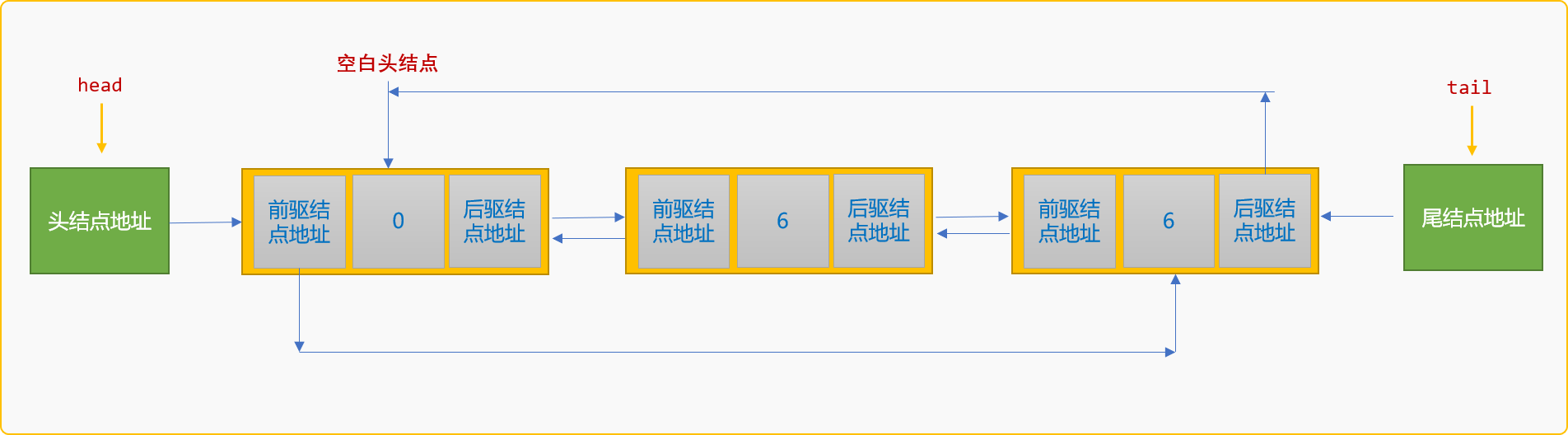• 链表初始化。
• 创建链表。
• 查找。
• 后插入、前插入。
• 删除。
• ……

### 2.1 初始化

• `head``tail`指向空白头结点。
• `head`的前驱结点和`tail`的后驱结点也指向空白头结点。这个过程也可以放到创建链表时实现。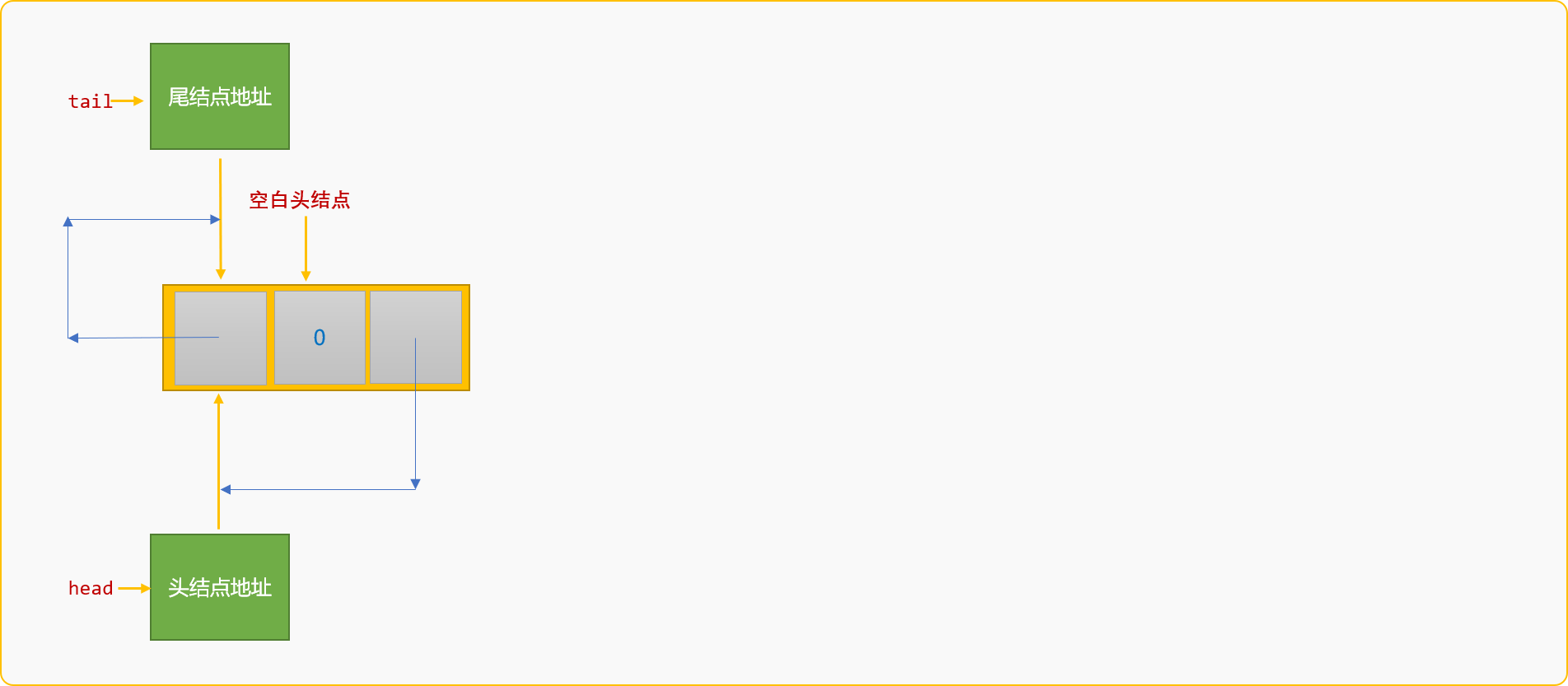``````class LinkList {
private:
//头指针
//尾指针
//链表的长度
int length;
public:
//构造函数
}
//初始化链表
//头指针存储空白头结点地址
//尾指针和头指针位置相同
//尾结点的后驱结点是头结点
//头结点的前驱结点是尾结点
}
//……其它函数
``````

### 2.2 创建链表

• 创建值为`7`的新结点`n`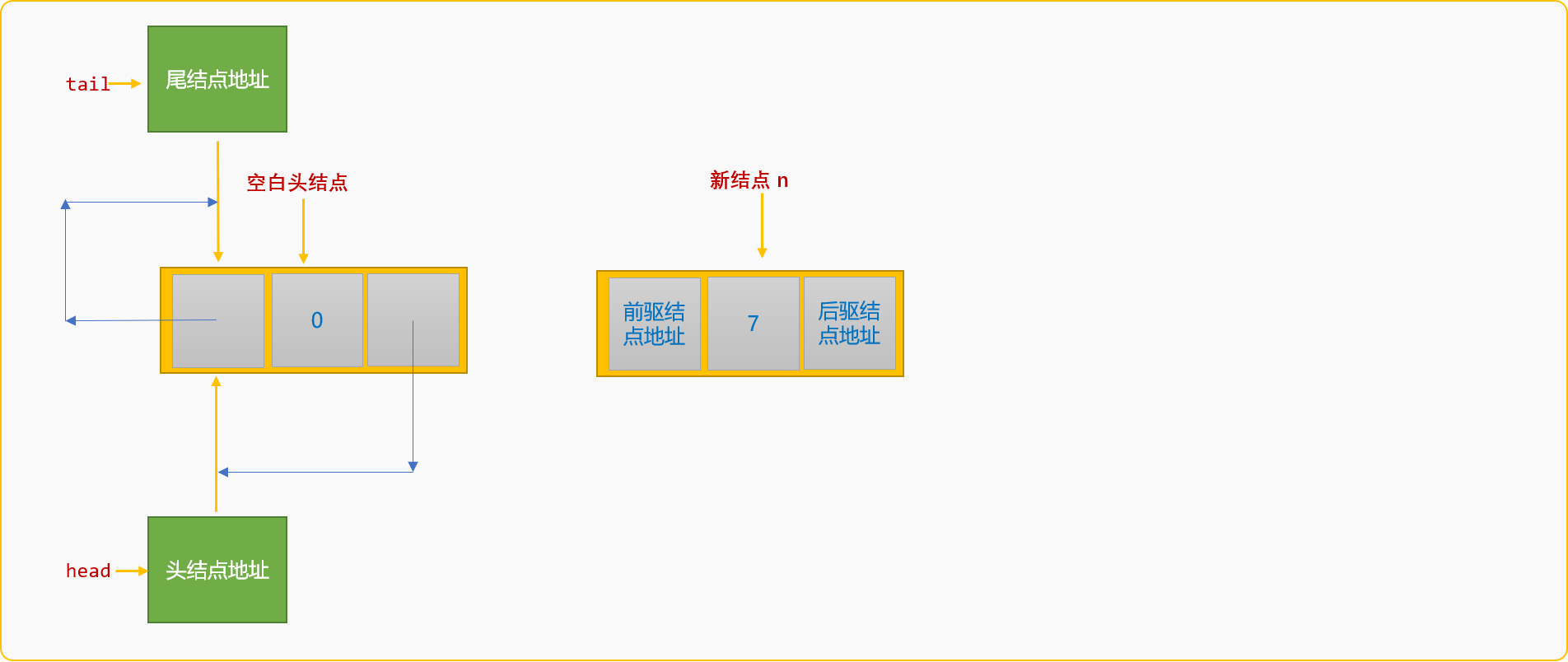• 设置新结点`n`的前驱结点为原尾结点。
``````n->pre=tail;
``````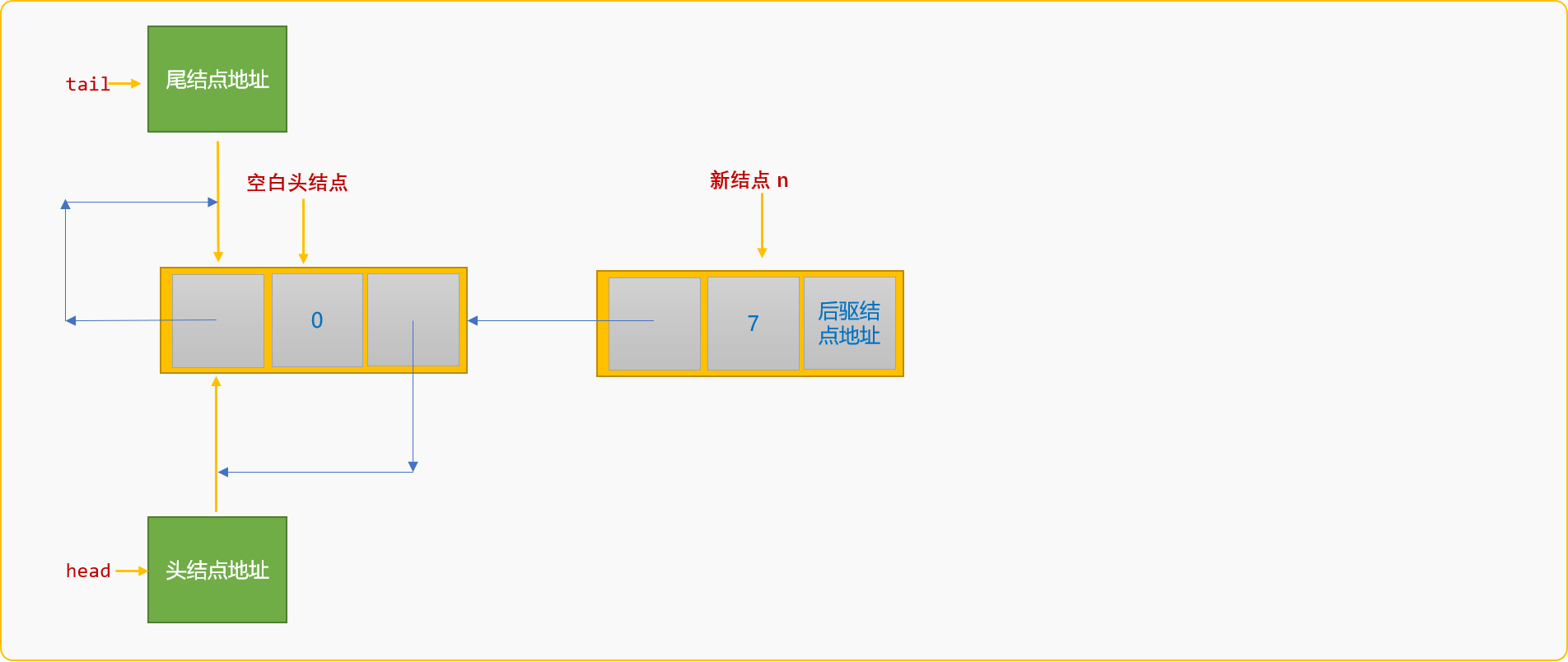• 设置新结点`n`的后驱结点为原尾结点的后驱结点。
``````n->next=tail->next;
``````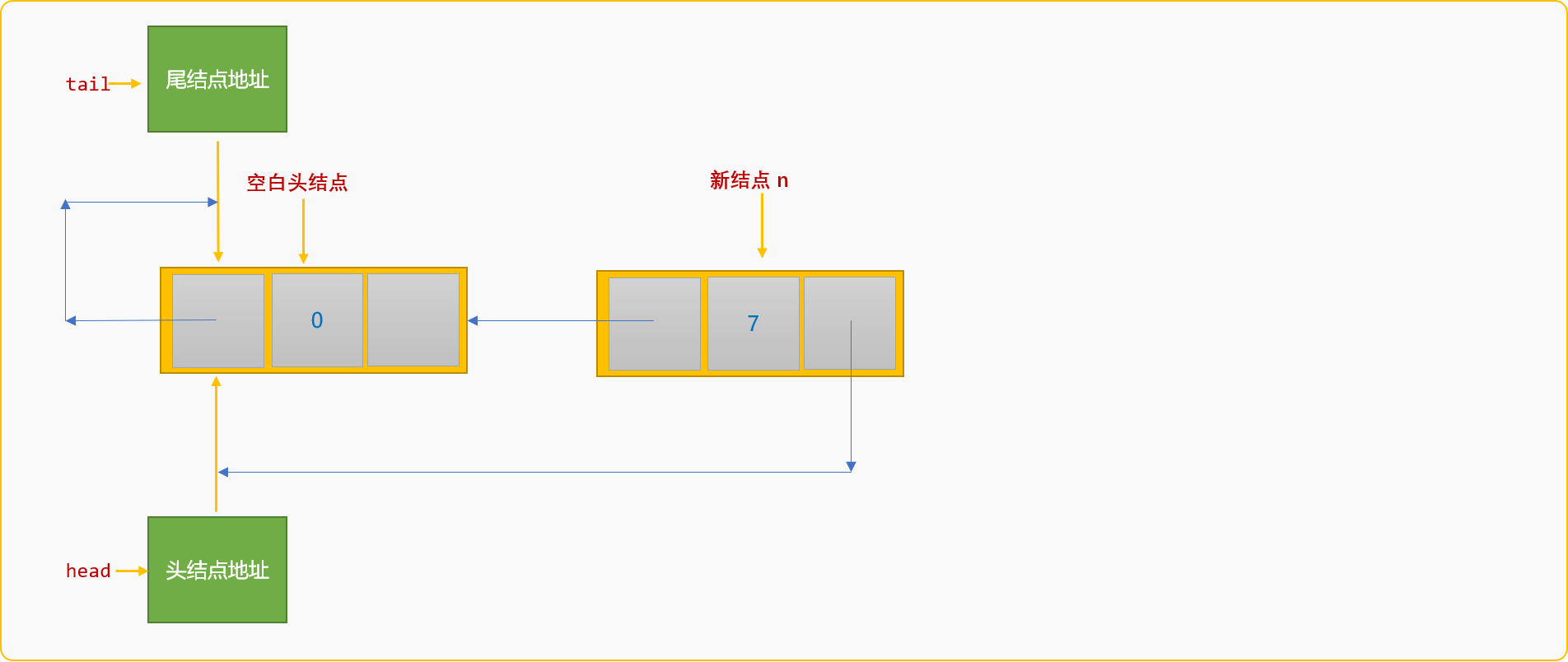• 设置原尾结点的后驱结点为新结点`n`
``````tail->next=n;
``````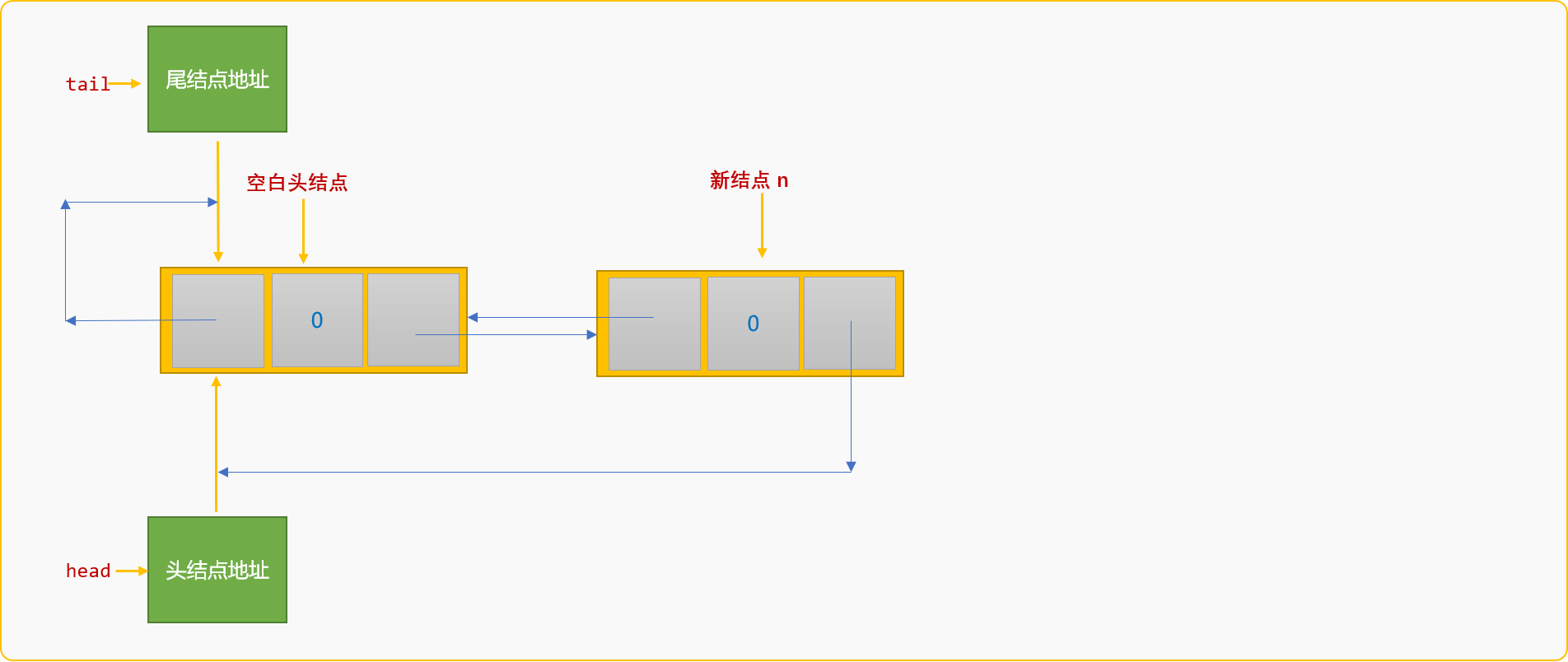• 新结点`n`成为新的尾结点。
``````tail=n;
``````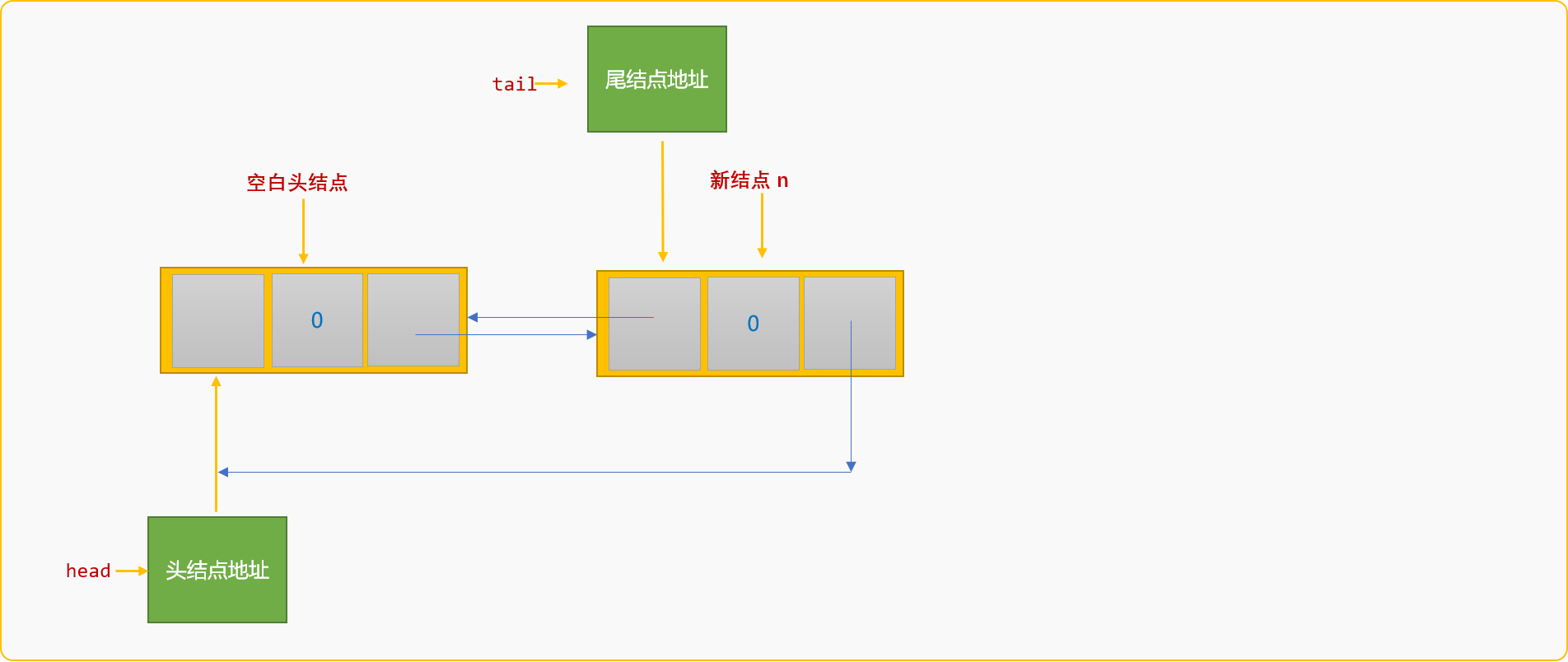• 设置头结点的前驱结点为新的尾结点。
``````head->pre=tail;
``````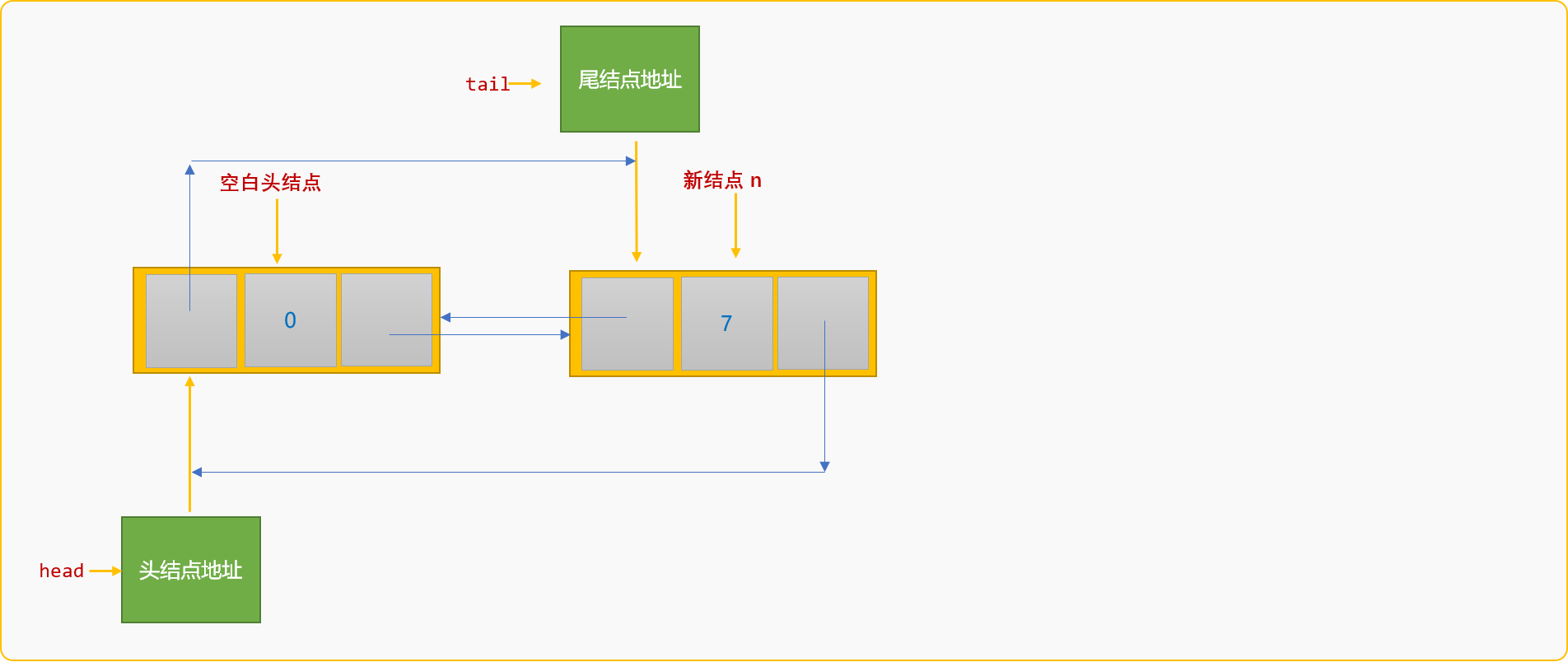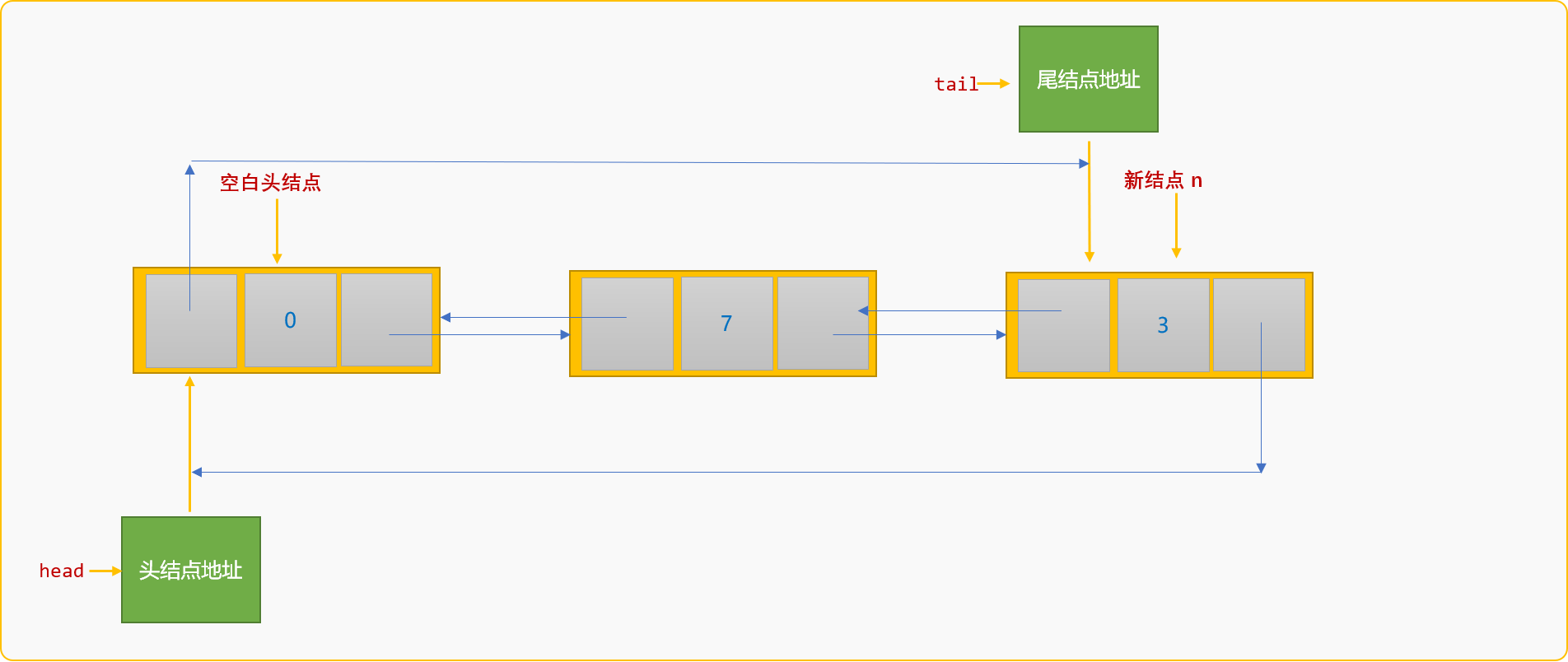• 新结点：先设置新结点的前驱和后驱结点的地址。新结点的相应存储位都是空白的，先设置前驱还是后驱不影响结果。
• 尾结点：修改原尾结点的后驱结点地址为新结点，原尾结点的使命完成后，再指定新结点为新的尾结点。
• 头结点：修改头结点的前驱地址为新尾结点。

``````//尾部插入方式创建链表
void createFromTail(int n) {
//头结点地址
//尾结点地址
tail=this->tail;
for(int i=; i<n; i++) {
//构建一个新结点
cout<<"请输入结点数据"<<endl;
cin>>newNode->data;
//原来的尾结点成为新结点的前驱结点
newNode->pre=tail;
//新结点的后驱结点为原来的尾结点的后驱结点
newNode->next=tail->next;
//原尾结点的后驱结点为新结点
tail->next=newNode;
//新结点成为新的尾结点
tail=newNode;
//头结点的前驱为新尾结点
}
this->tail=tail;
}
``````

``````int main(int argc, char** argv) {
list.createFromTail(2);
//没删除之前
cout<<"显示创建结果:"<<endl;
cout<<"从头结点向尾结点方向搜索："<<endl;
cout<<"从尾结点向头结点方向搜索 ："<<endl;
cout<<tail->data<<endl;
cout<<tail->pre->data<<endl;
cout<<"从尾结点的后驱信息位得到头结点信息 ："<<endl;
return ;
}
``````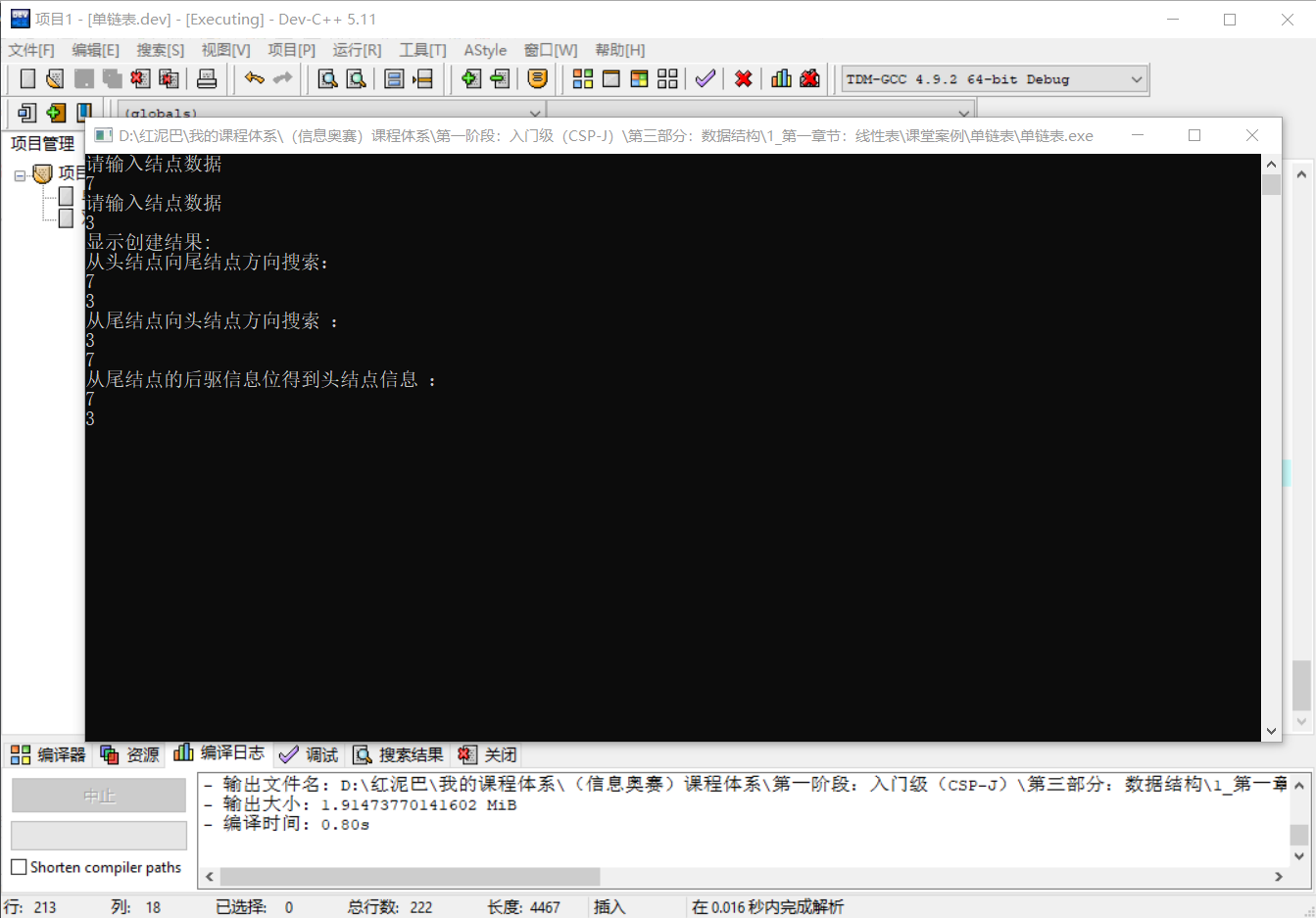### 2.3 查找

`双向循环链表`的头尾是相连的，其查询方案可以有多种变化：

• 按位置查找： 按位置查找建议从头结点开始。
• 按值查找： 按值查找可以从头结点或从尾结点开始。
• 查询所有： 查询所有可以从头结点也可以从尾结点开始。

### 2.3.1 按位置查找

``````//按位置查询结点（从头部扫描到尾部）
LinkNode * findNodeByIndex(int index) {
int j=;
if(index==j)
//如果 index 值为 0 ，返回空白头结点
return p;
//个数据结点
p=p->next;
//设置个数据结点的编号为 1 ，当然这不是，可以根据自己的需要设置编号
j=1;
while(p!=this->head && j<index) {
p=p->next;
j++;
}
if(j==index)return p;
else return NULL;
}
``````

#### 2.3.2 按值查找

• 头结点向尾结点方向查找。
``````//按值查询结点
LinkNode * findNodeByVal(dataType val) {
//从个数据结点开始查找
//当 p 再次指向头结点结束查找，这也空白结点存在的意义
while(p!=this->head && p->data!=val ) {
p=p->next;
}
return p;
} else {
return NULL;
}
}
``````
• 尾结点向头结点方向查找。
``````LinkNode * findNodeByValFromTail(dataType val) {
//从尾结点开始查找
while(p!=this->head && p->data!=val ) {
//向头结点方向
p=p->pre;
}
return p;
} else {
return NULL;
}
}
``````

#### 2.3.3 查询所有

• 从头结点向尾结点方向查询所有结点。
``````void showSelf() {
//得到个数据结点
cout<<p->data<<"\t";
p=p->next;
}
}
``````
• 从尾结点向头结点方向查询所有结点。
``````void showSelf_() {
if(this->tail==NULL)return;
cout<<p->data<<"\t";
p=p->pre;
}
}
``````

### 2.4 插入

#### 2.4.1 后插入

• 找到已知结点`p`，创建新结点`n`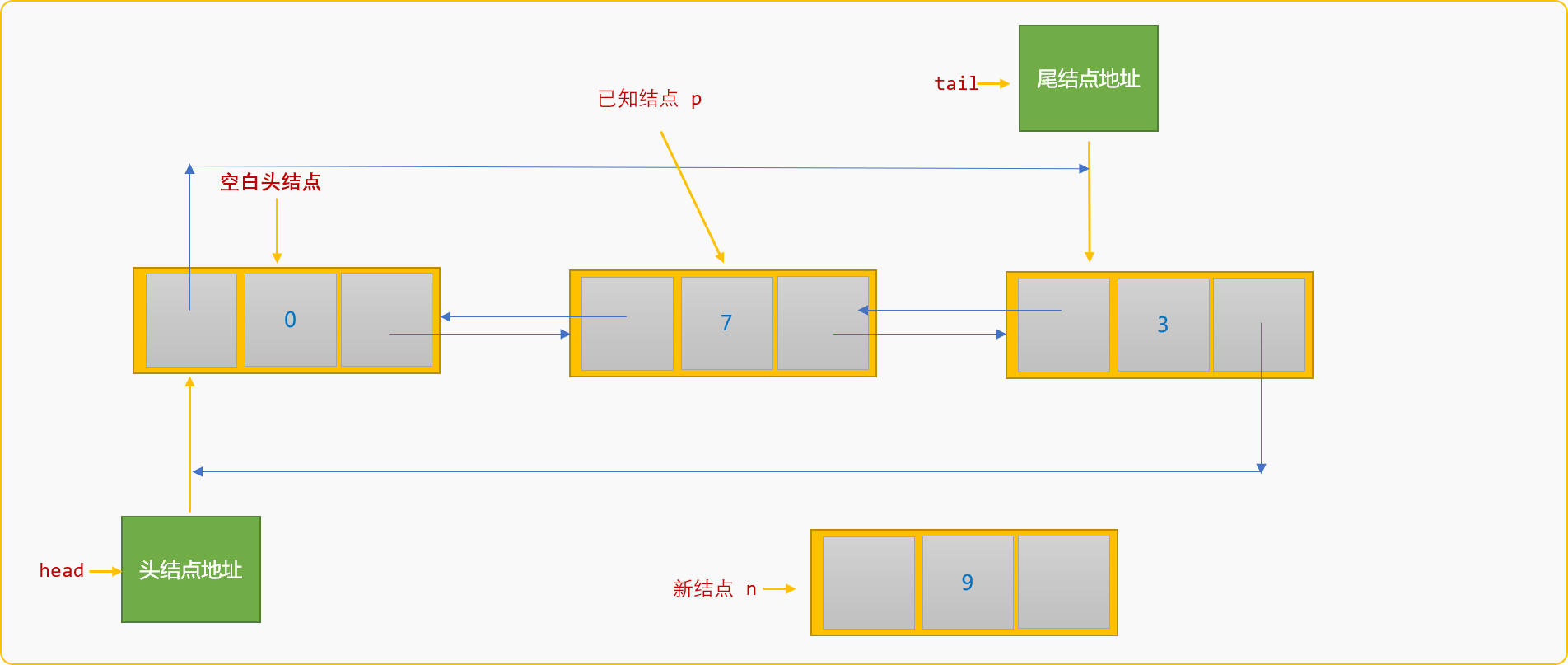• 设置`n`结点的前驱结点为已知结点`p`，设置其后驱结点为已知结点`p`的后驱结点。
``````n->pre=p;
n->next=p->next;
``````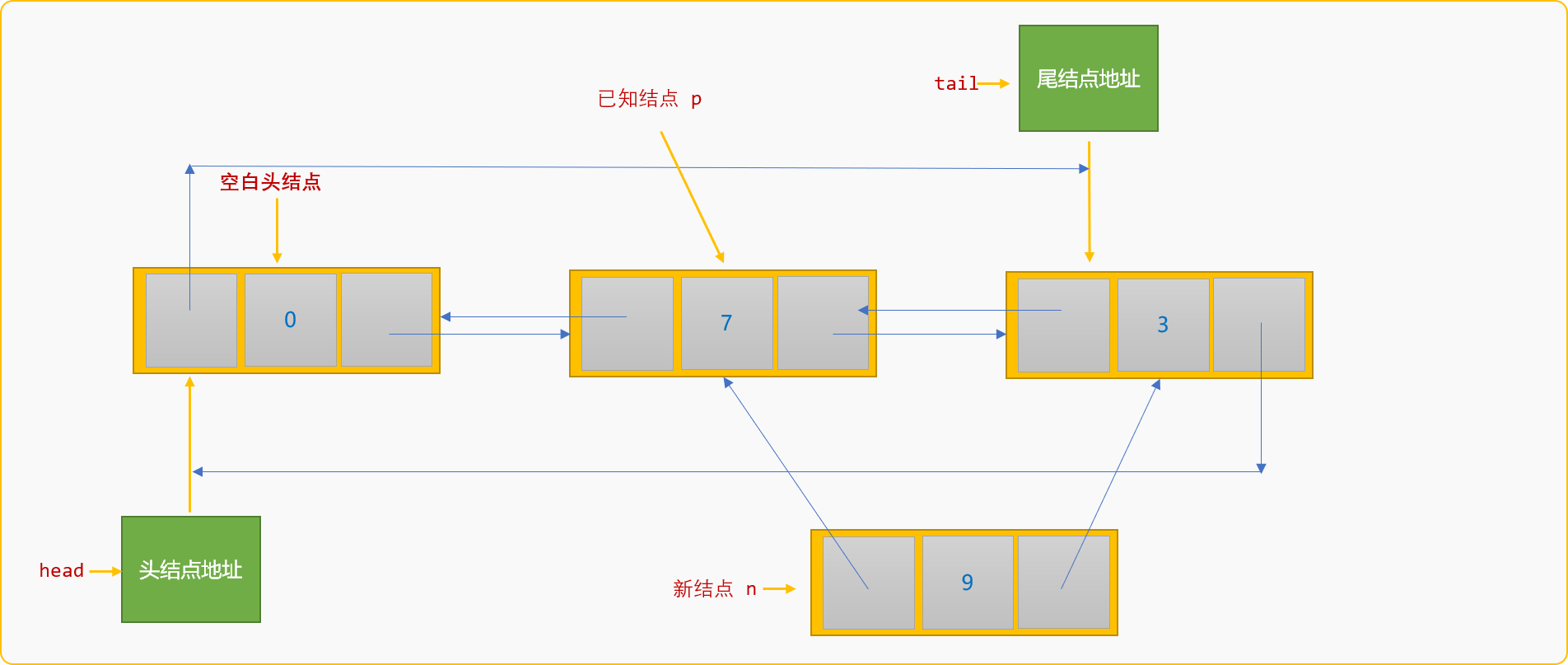• 设置`p`结点的后驱结点为`n`结点。
``````p->next=n;
``````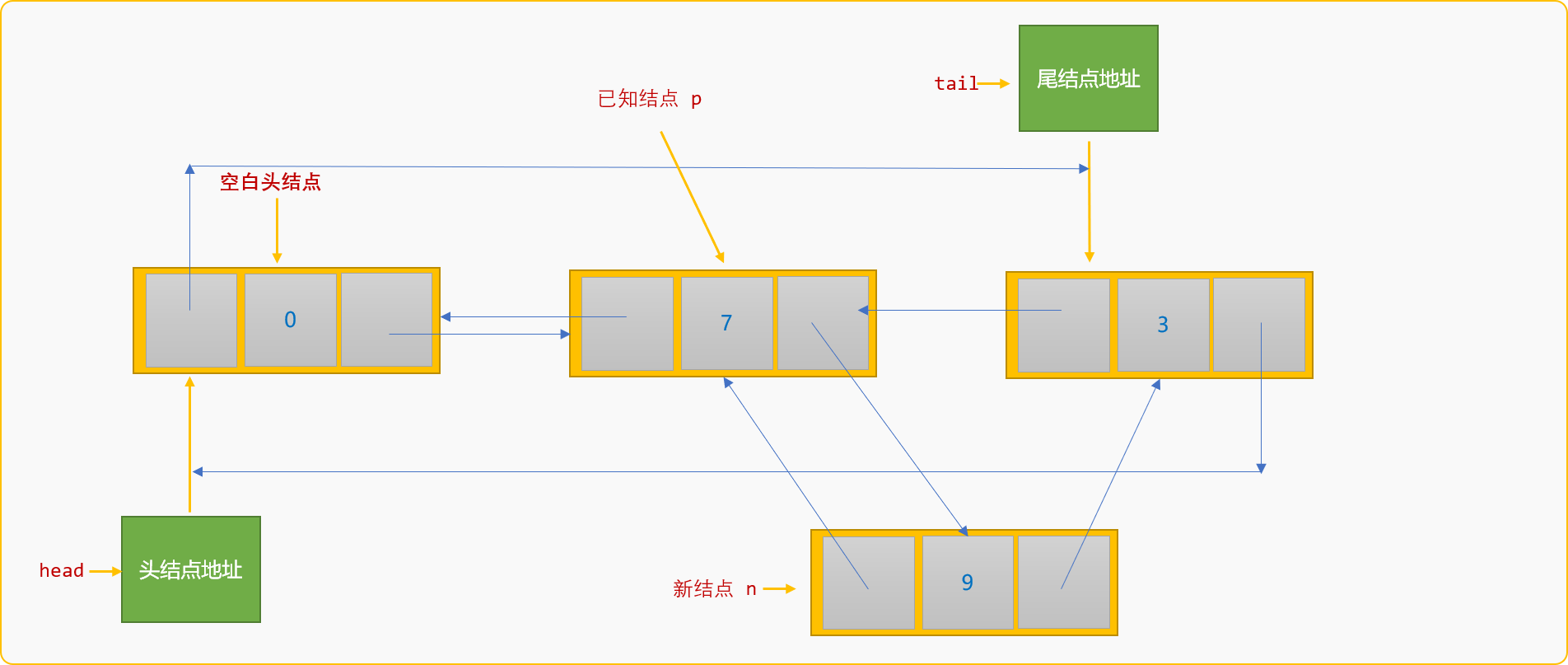• 设置结点`n`为其后驱结点的前驱结点。
``````n->next->pre=n;
``````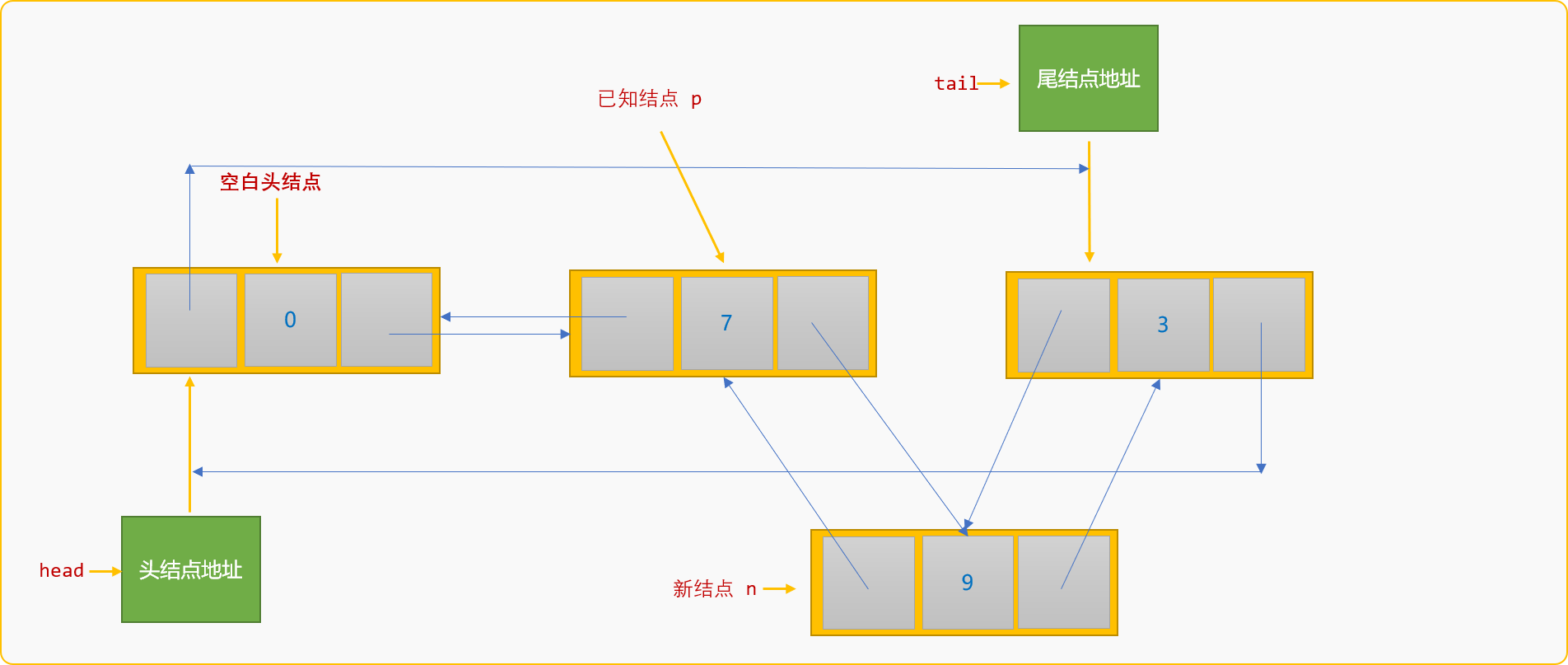``````	//后插入
int instertAfter(dataType val,dataType data) {
//按值查找到结点
if (p==NULL) {
//结点不存在，返回 false
return false;
}
//创建新结点
n->data=data;
//设置 p 结点为新结点的前驱结点
n->pre=p;
//新结点的后驱结点为已知结点 p 的后驱结点
n->next=p->next;
//已知结点的后驱结点为新结点
p->next=n;
//已知结点的原后驱结点的前驱结点为新结点
n->next->pre=n;
return true;
}
``````

``````int main(int argc, char** argv) {
//创建 7,3 两个结点
list.createFromTail(2);
//在结点 7 后面插入值为 9 的结点
list.instertAfter(7,9);
list.showSelf();
return ;
}
``````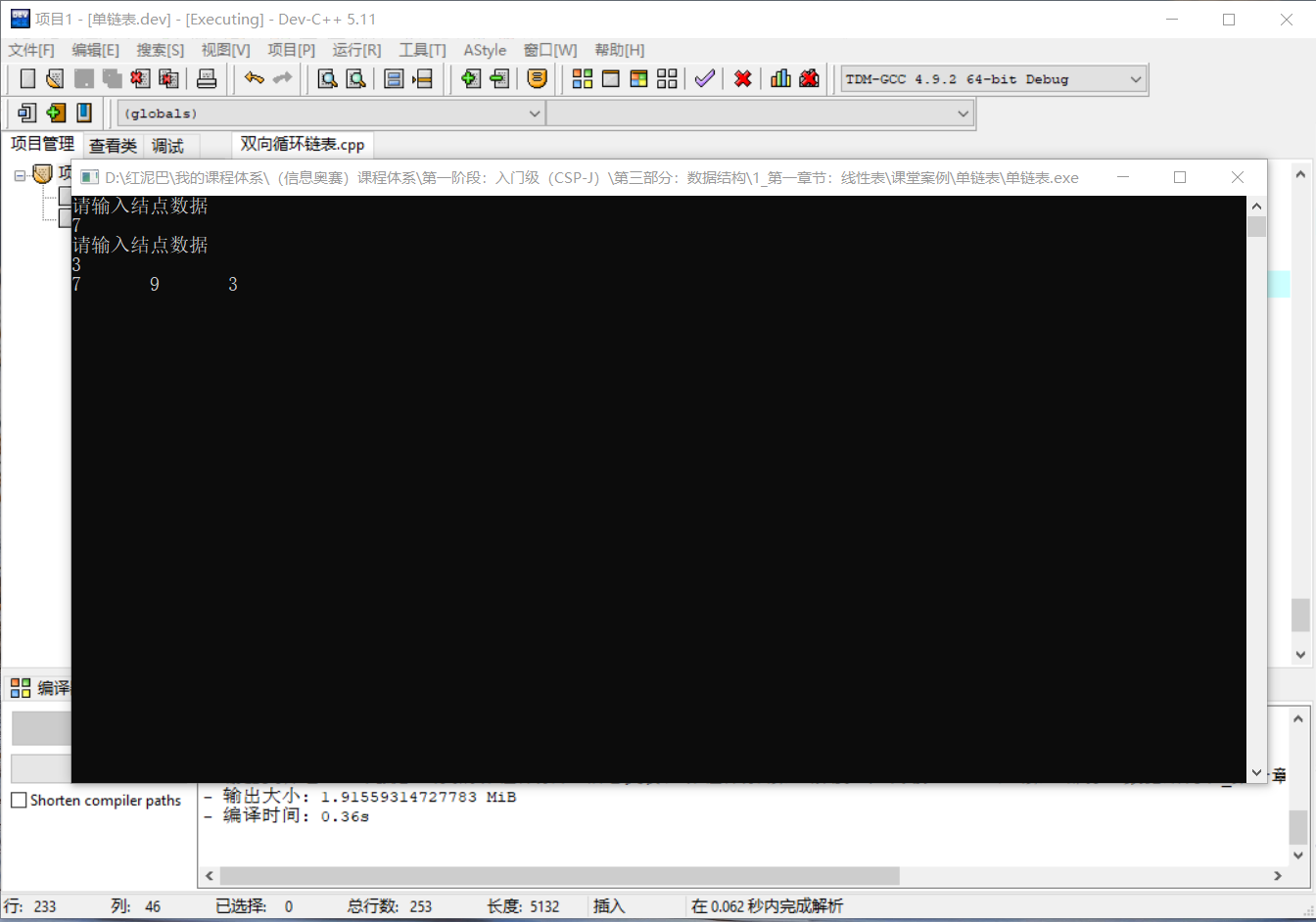#### 2.4.2 前插入

• 找到已知结点`p`，创建新结点`n`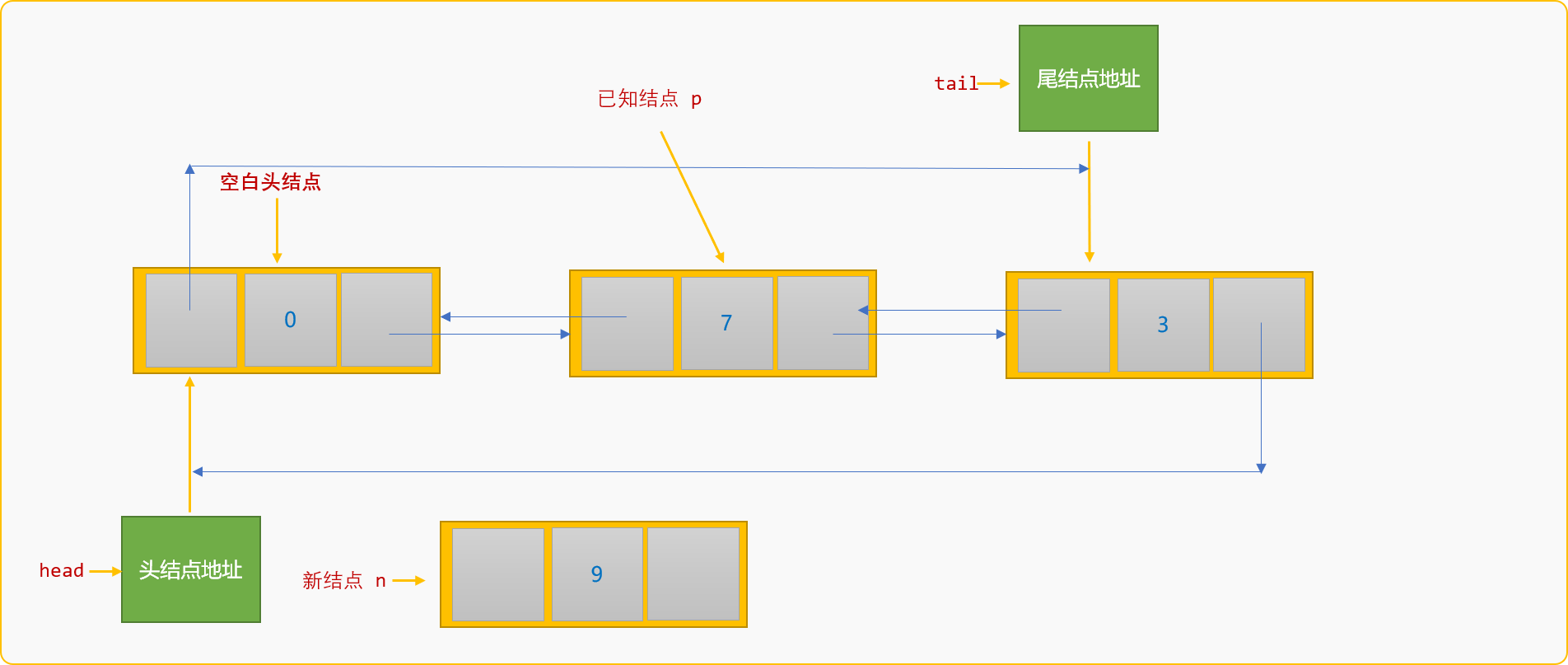• 设置结点`n`的前驱结点为`p`的前驱结点，设置其后驱结点为`p`结点。
``````n->pre=p->pre;
n-next=p;
``````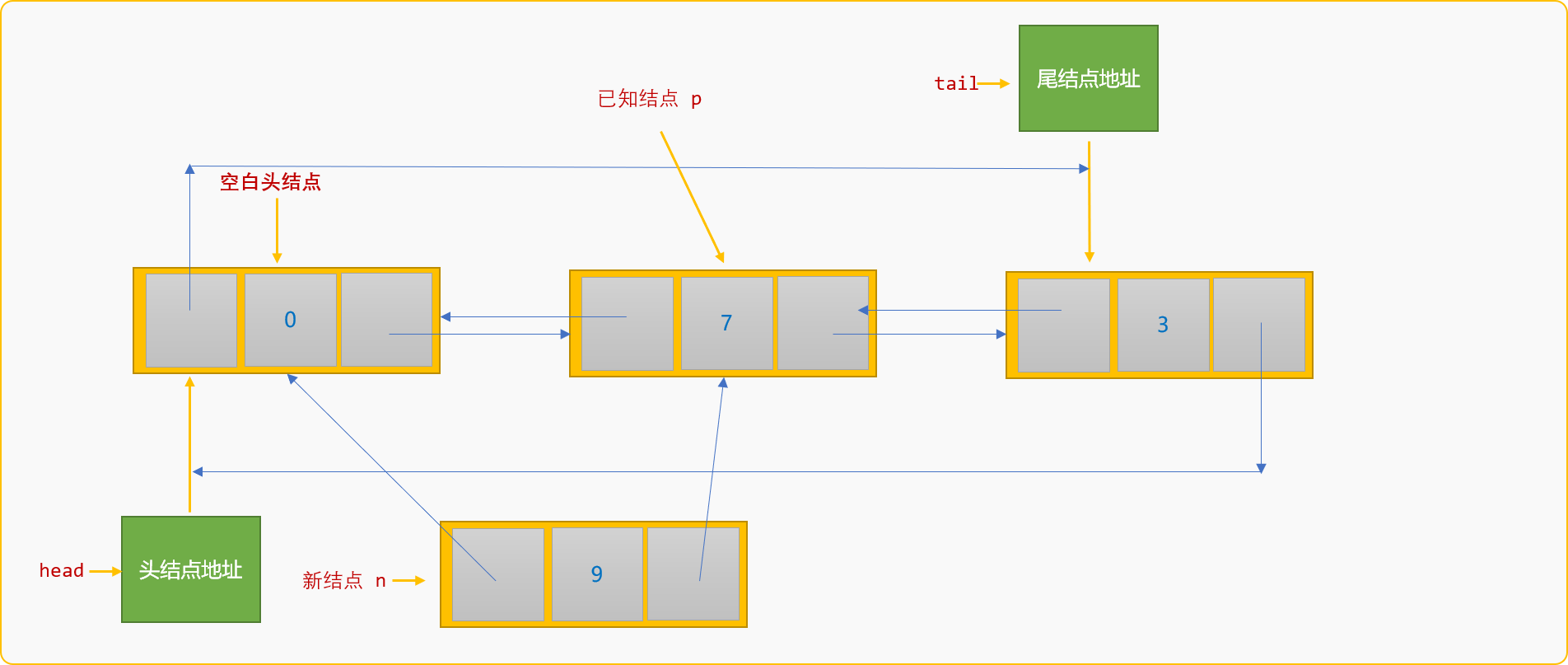• 设置`p`结点的前驱结点的后驱结点为`n`
``````p->pre->next=n;

n->pre->next=n;
``````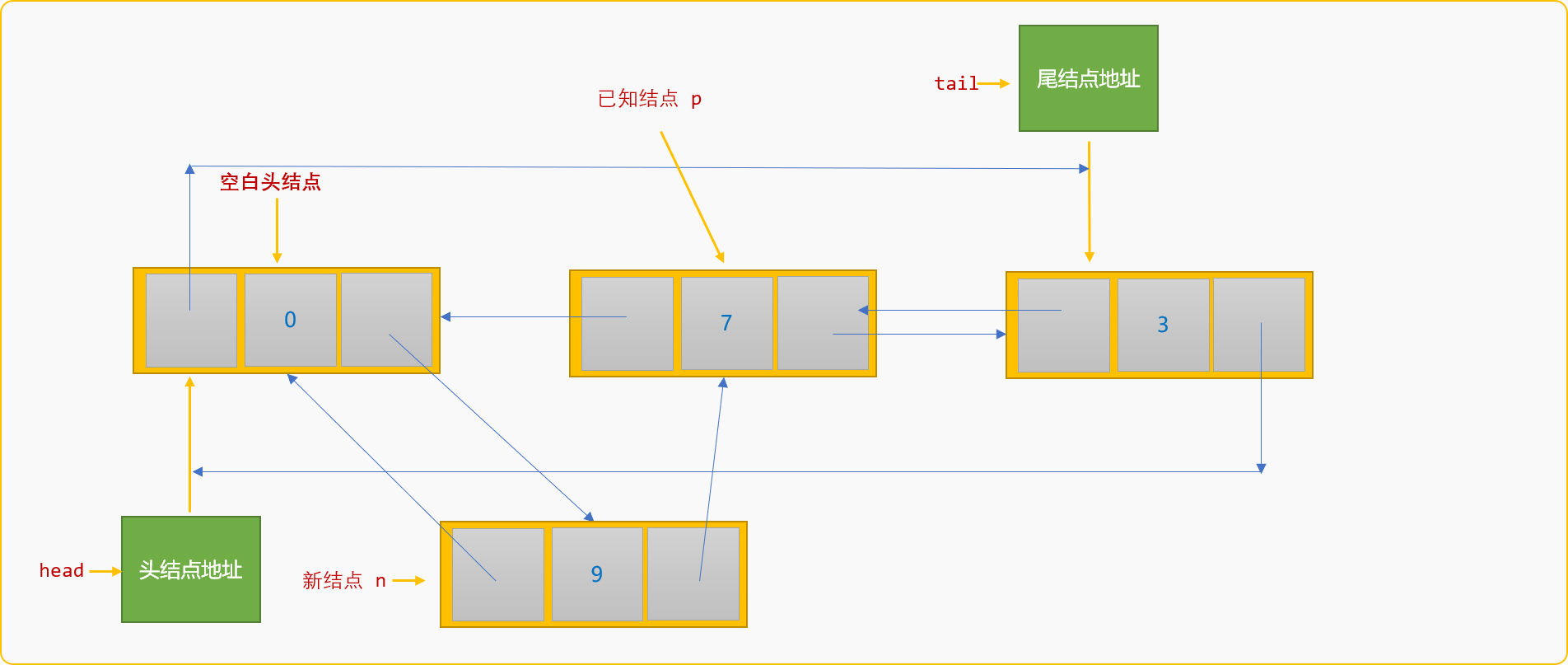• 设置`p`结点的前驱结点为`n`结点。
``````p->pre=n;
``````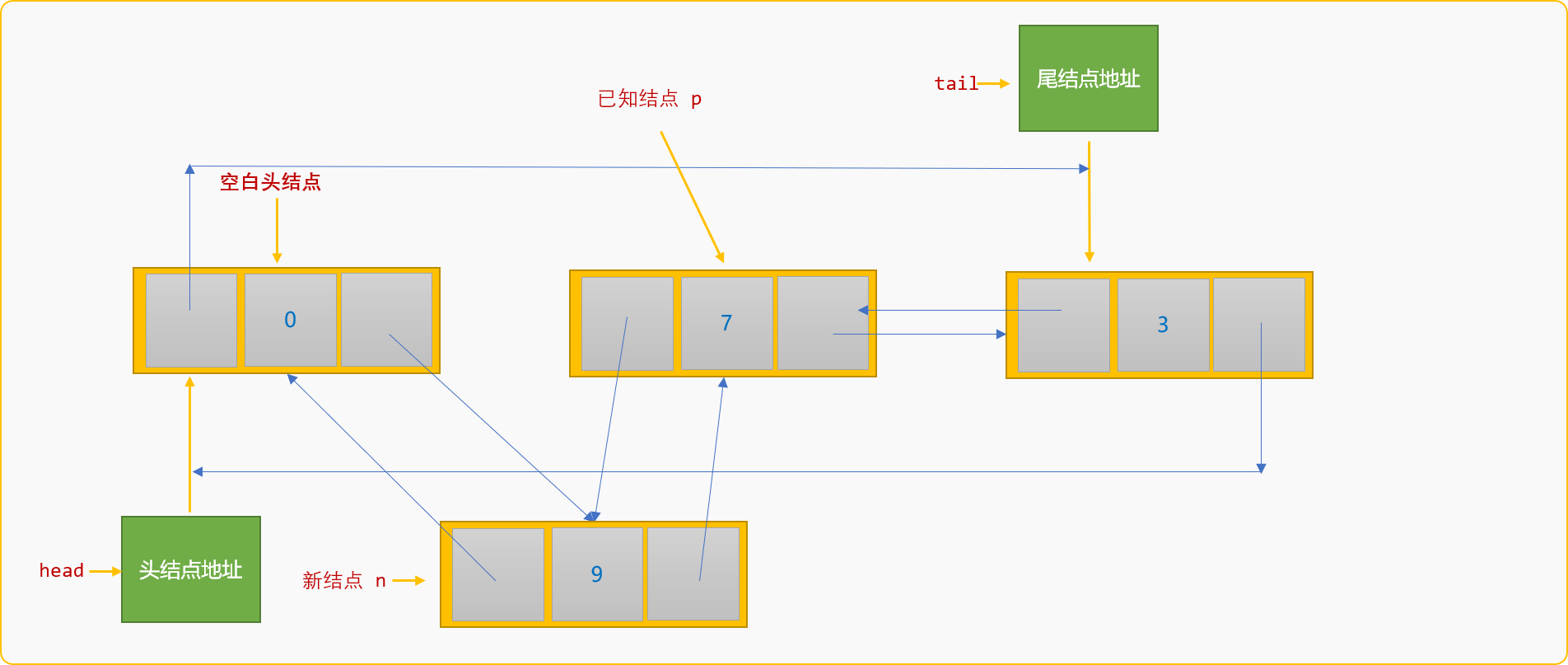``````//前插入
int insertBefore(dataType val,dataType data) {
//按值查找到结点
if (p==NULL)
return false;
//查找前驱结点
while(p1->next!=p) {
p1=p1->next;
}
//构建新结点
n->data=data;
//新结点的后驱为 p 结点
n->next=p;
//新结点的前驱为 p 的前驱
n->pre=p->pre;
//p 的前驱结点的后驱结点为 n
p->pre->next=n;
//p 的前驱结点为 n
p->pre=n;
return true;
}
``````

``````int main(int argc, char** argv) {
//创建 7,3 两个结点
list.createFromTail(2);
//在值为 7 的结点前面插入值为 9 的结点
list.insertBefore(7,9);
list.showSelf();
return ;
}
``````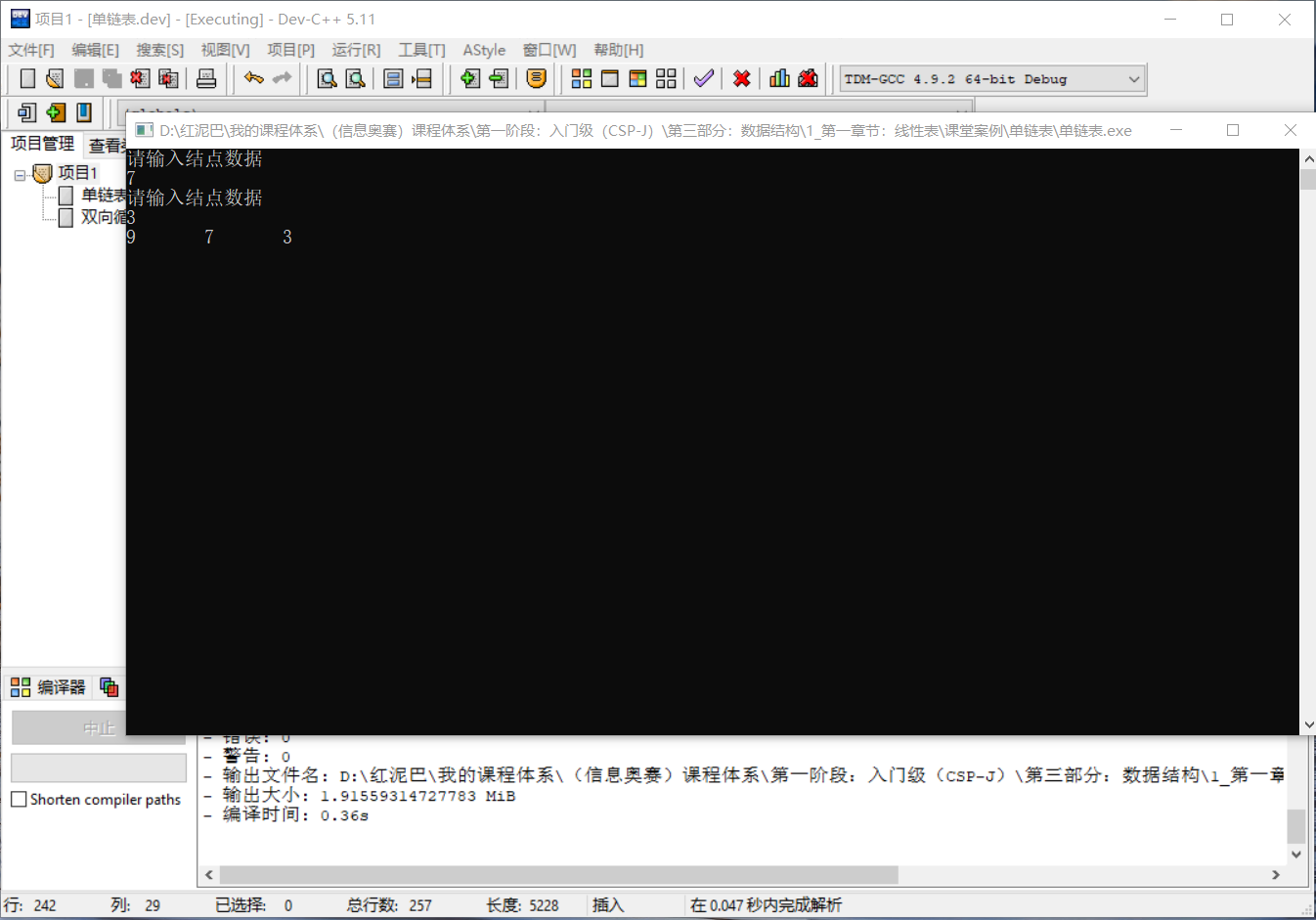### 2.5 删除

#### 2.5.1 删除结点

• 查找到要删除的结点`p`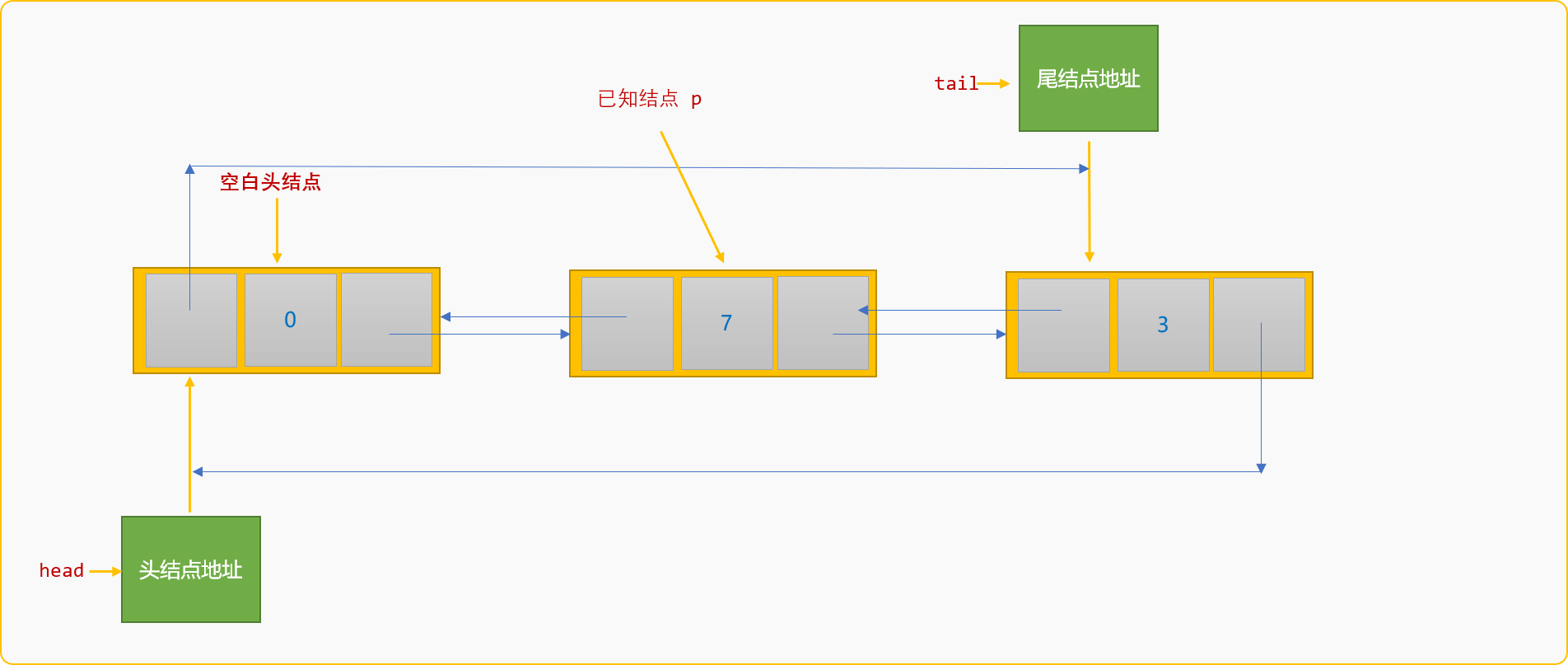• 找到结点`p`的前驱结点，设置其后驱结点为`p`的后驱结点。
``````p->pre->next=p->next;
``````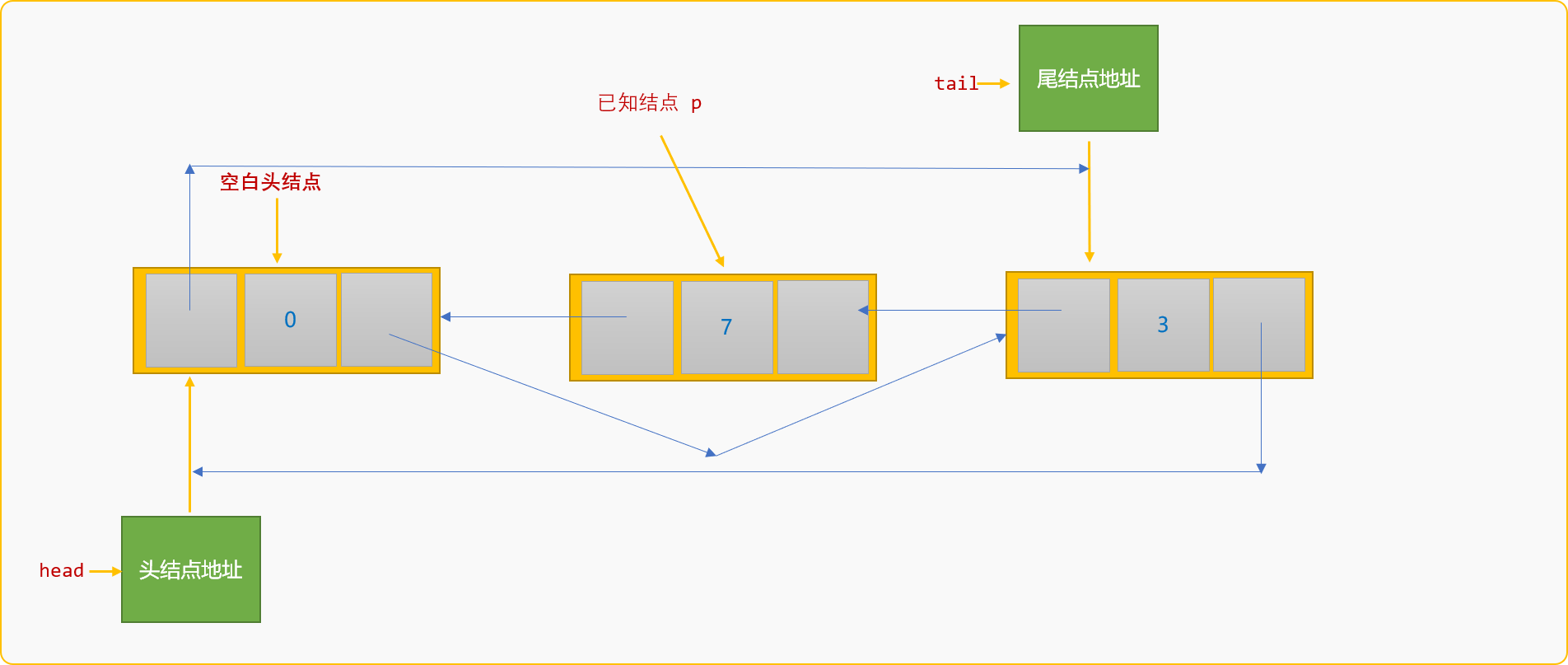• 找到`p`结点的后驱结点，设置其前驱结点为`p`结点的前驱结点。删除`p`结点。
``````p->next->pre=p->pre;
delete p;
``````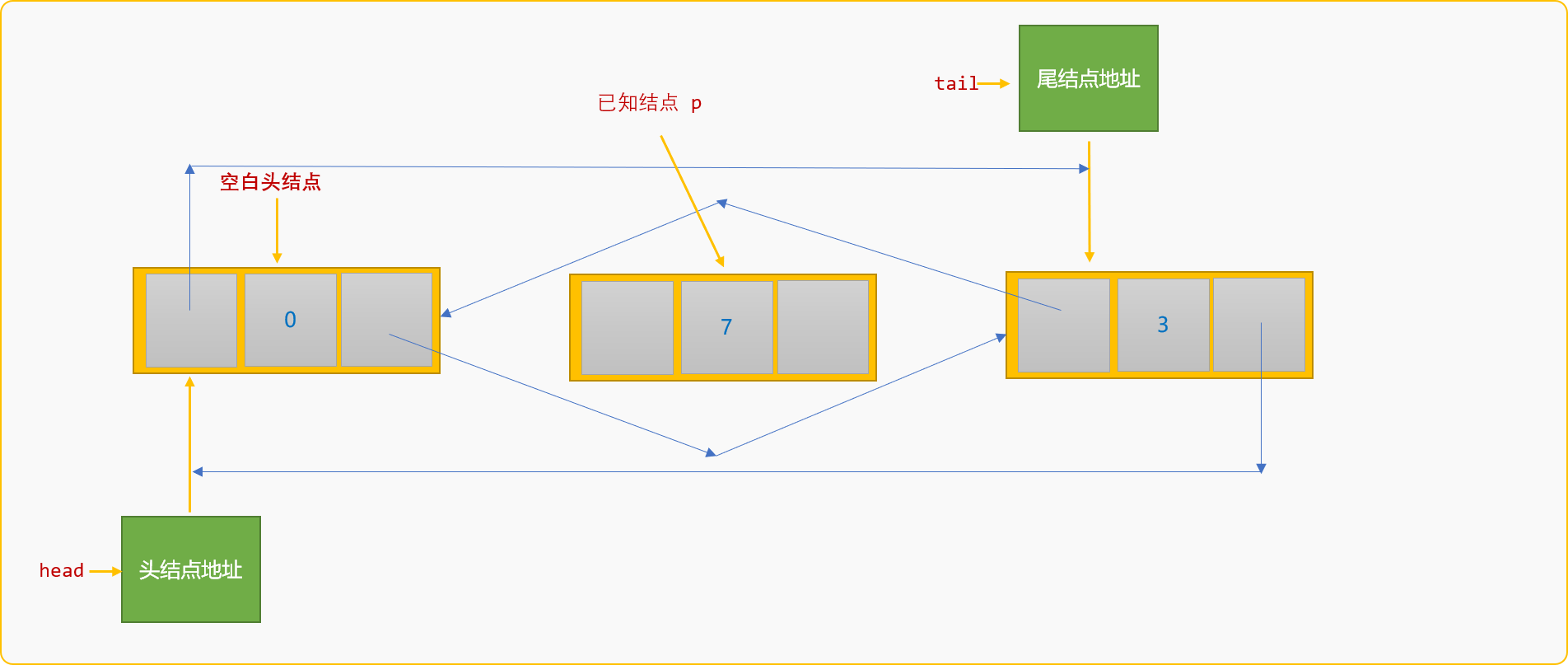``````int delNode(dataType data) {
//按值查找到要删除的结点
if (p==NULL)return false;
//设置 p 的前驱结点的后驱结点
p->pre->next=p->next;
p->next->pre=p->pre;
delete p;
return true;
}
``````

``````LinkList list {};
//创建 {7,3,9} 3个结点
list.createFromTail(3);
list.delNode(3);
list.showSelf();
``````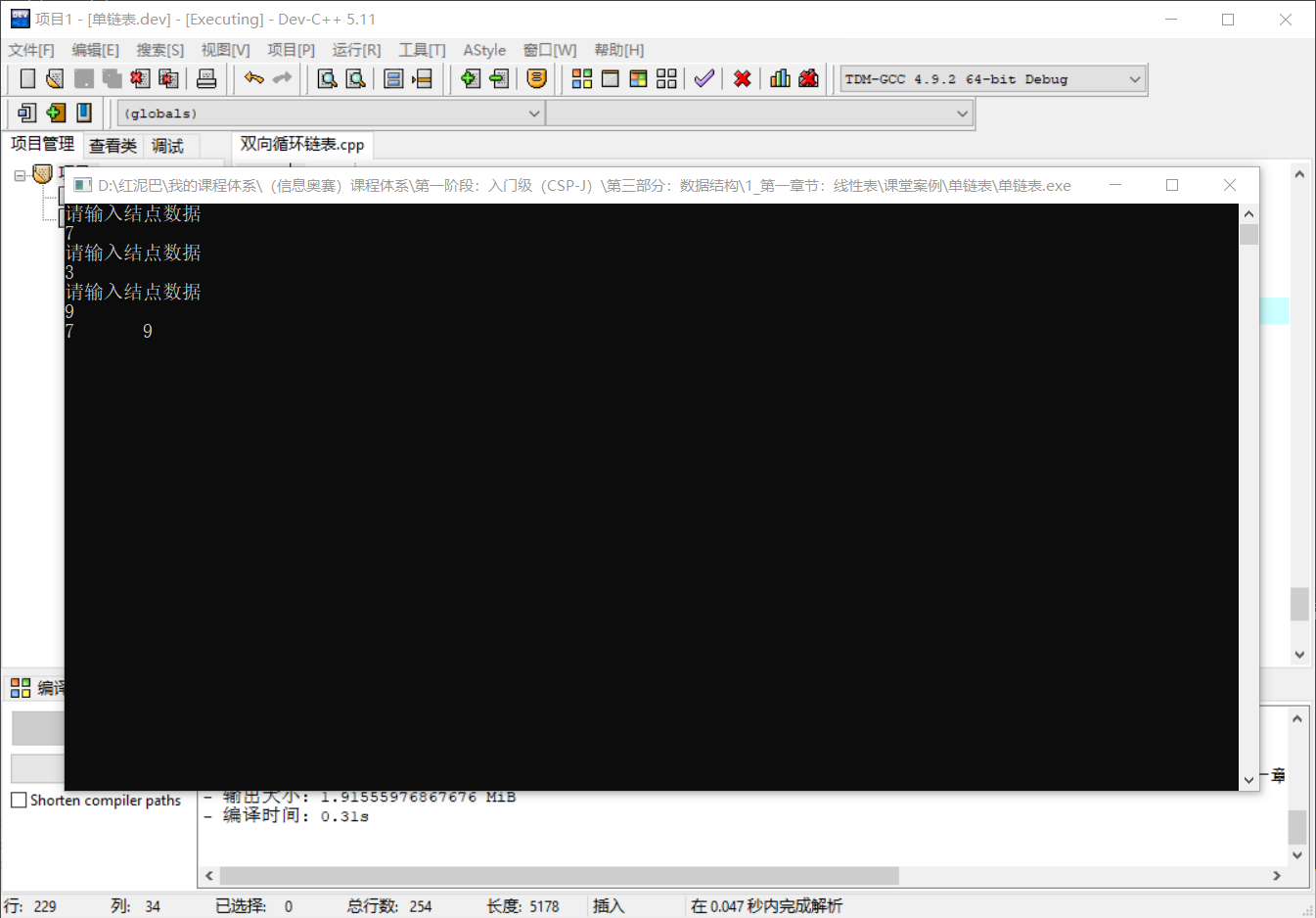#### 2.5.2 删除所有结点

``````void delAll() {
//临时结点
//保留删除结点的后驱结点
p1=p->next;
delete p;
p=p1;
}
}
``````

## 3. 算法案例

`界定数列`的要求：对于一个无序数列，首先在数列中找出一个基数，然后以基数为分界点，把小于基数的数字放在基数前面，反之放在后面。

### 3.1 演示流程

• 已知的无序数列：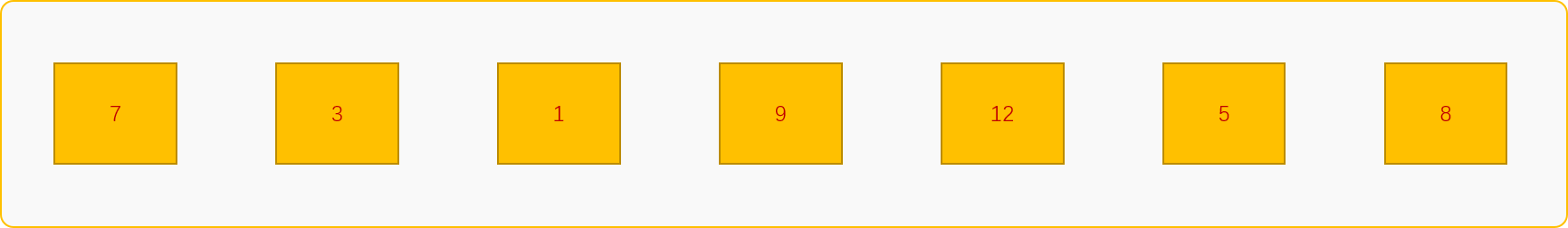• 选择基数。这里选择个数字 `7` 作为基数。保存在临时变量 `tmp`中。声明 `2` 个变量 `left``right`，分别指向个数据和后一个数据。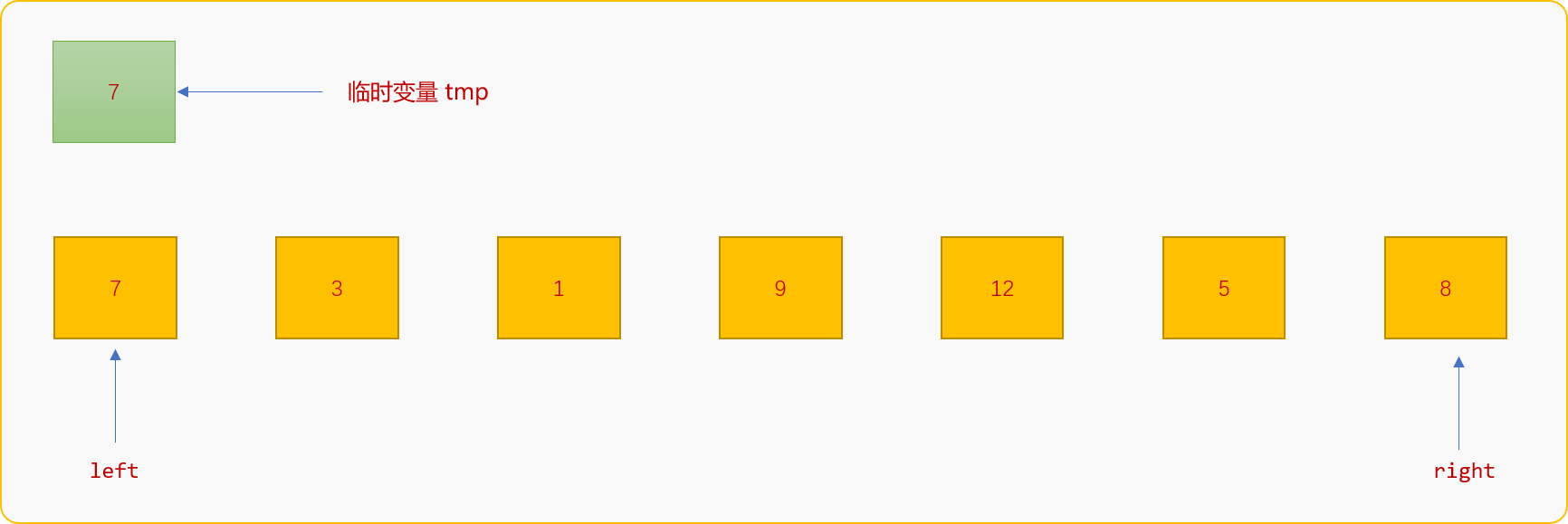• 从 `right`位置开始扫描整个数列，如果 `right`位置的数字大于 `tmp`中的值，则继续向左移动`right`指针直到遇到比 `tmp`中值小的数字，然后保存到 `left`位置。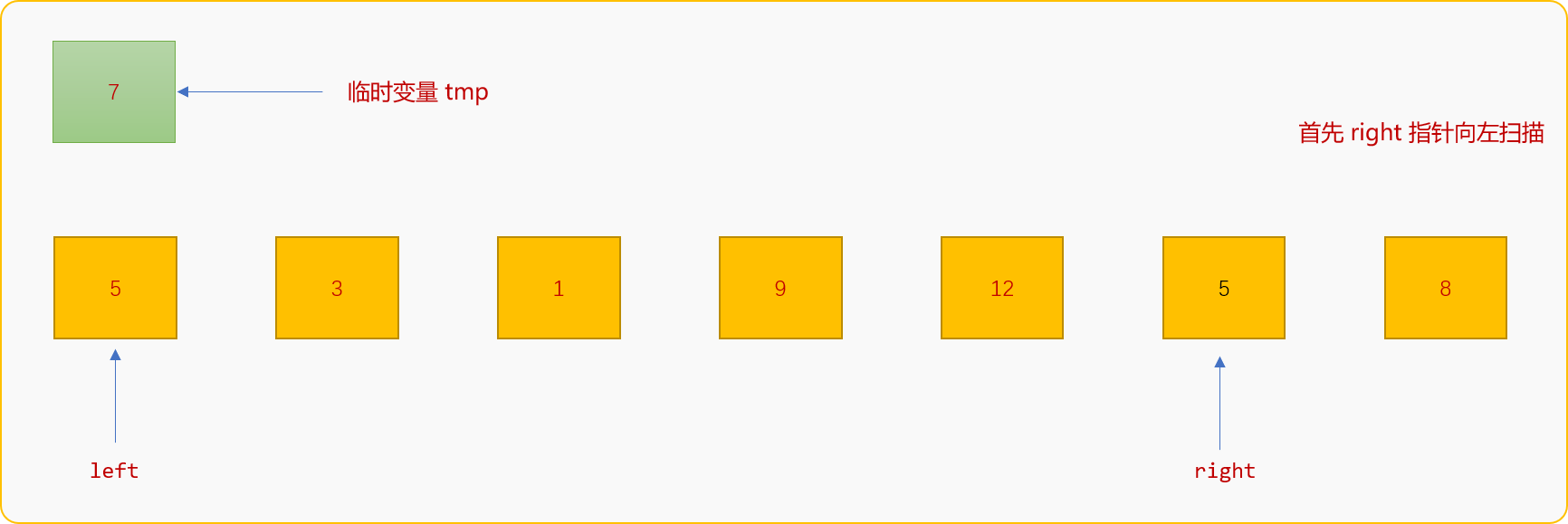• `left`指针的工作要求：当所处位置的数字比`tmp`值小时，则向右边移动直到遇到比`tmp`值大的数字，然后保存至`right`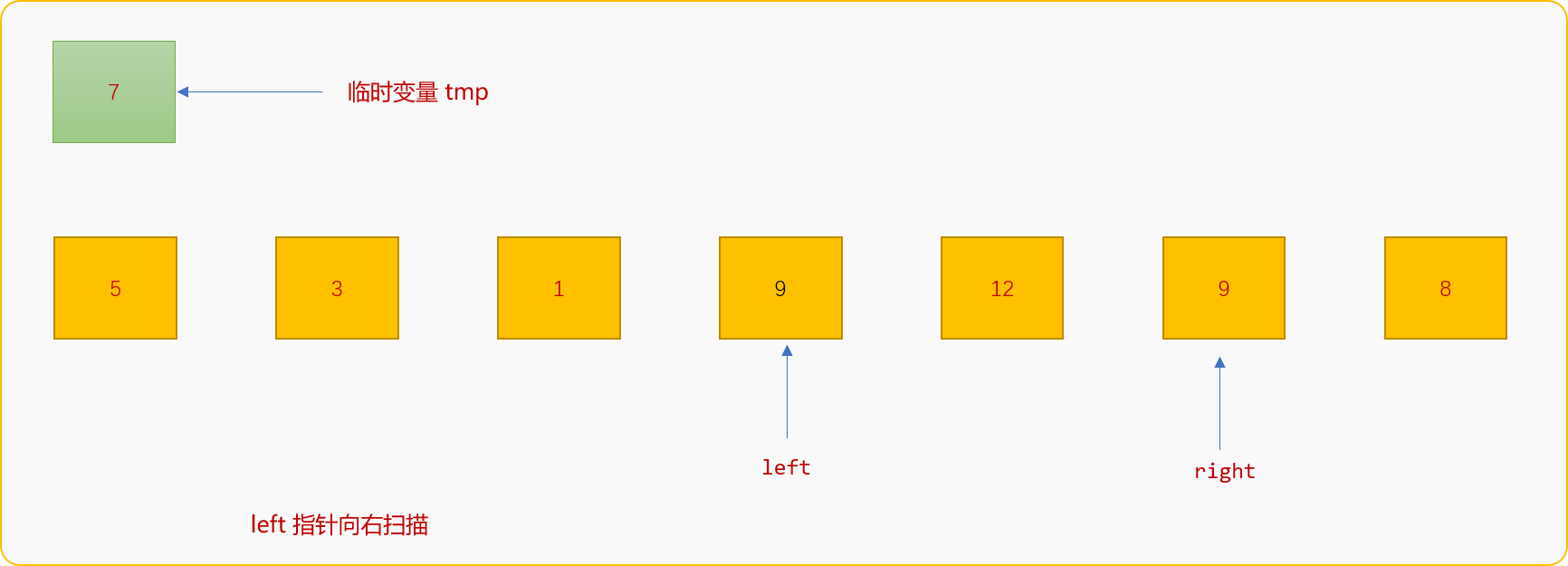• 重复上述过程，直到 `left``right`指针重合。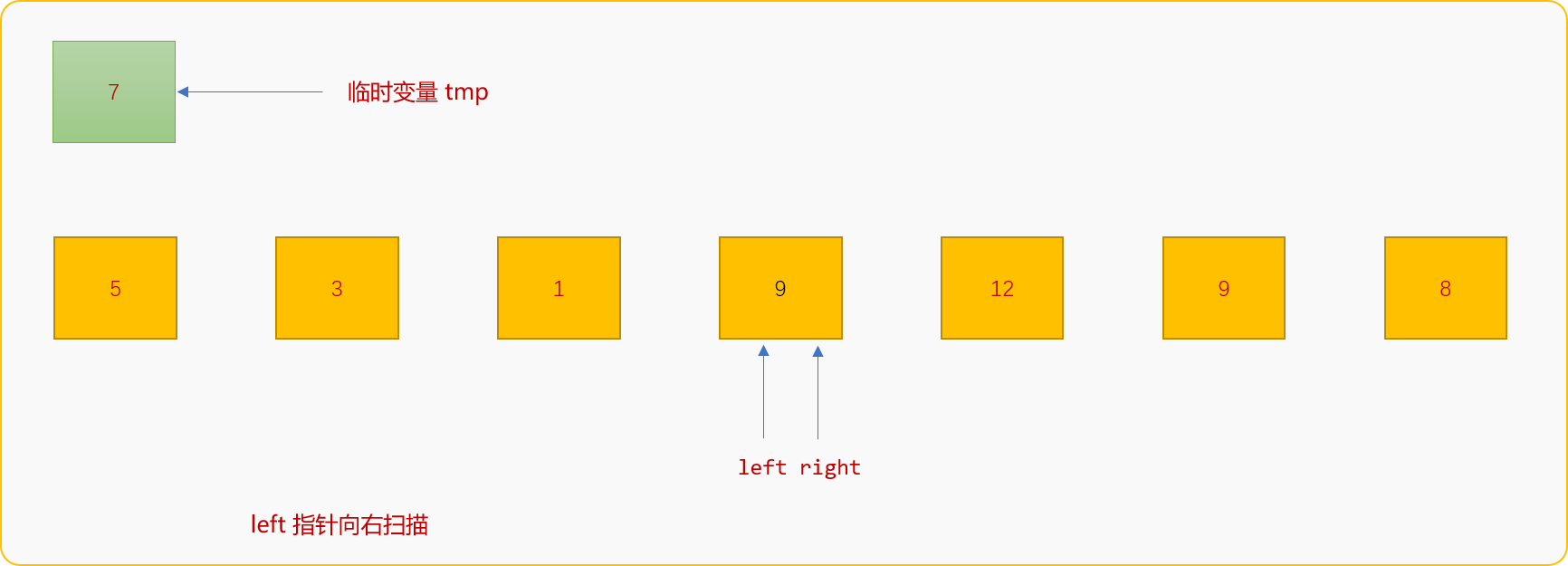• 后把`tmp`中的值复制到`left``right`指针后所指向的位置。终实现以数字`7`界定整个数列。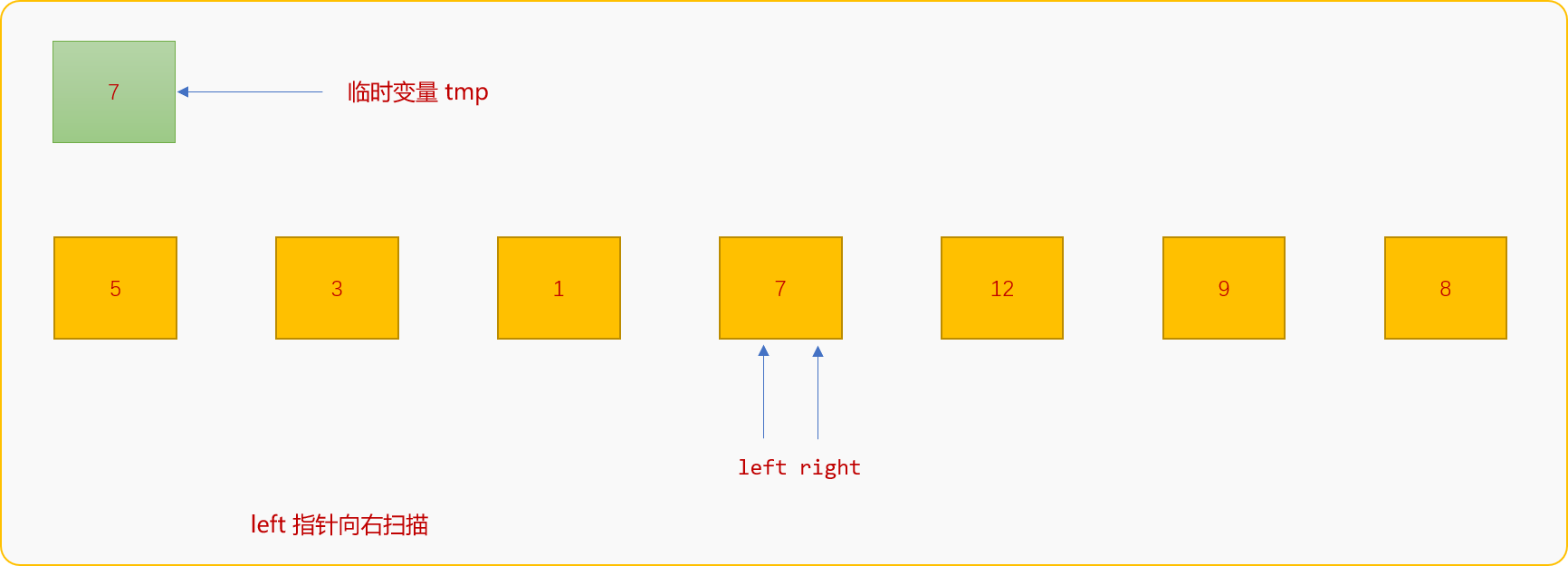### 3.2 算法实现

• 初始化链表，并以尾部插入方式（保证数列的逻辑顺序和物理顺序一致）创建数列`{7,3,1,9,12,5,8}`
``````int main(int argc, char** argv) {
list.createFromTail(7);
//没删除之前
cout<<"显示创建结果:"<<endl;
list.showSelf();
return ;
}
``````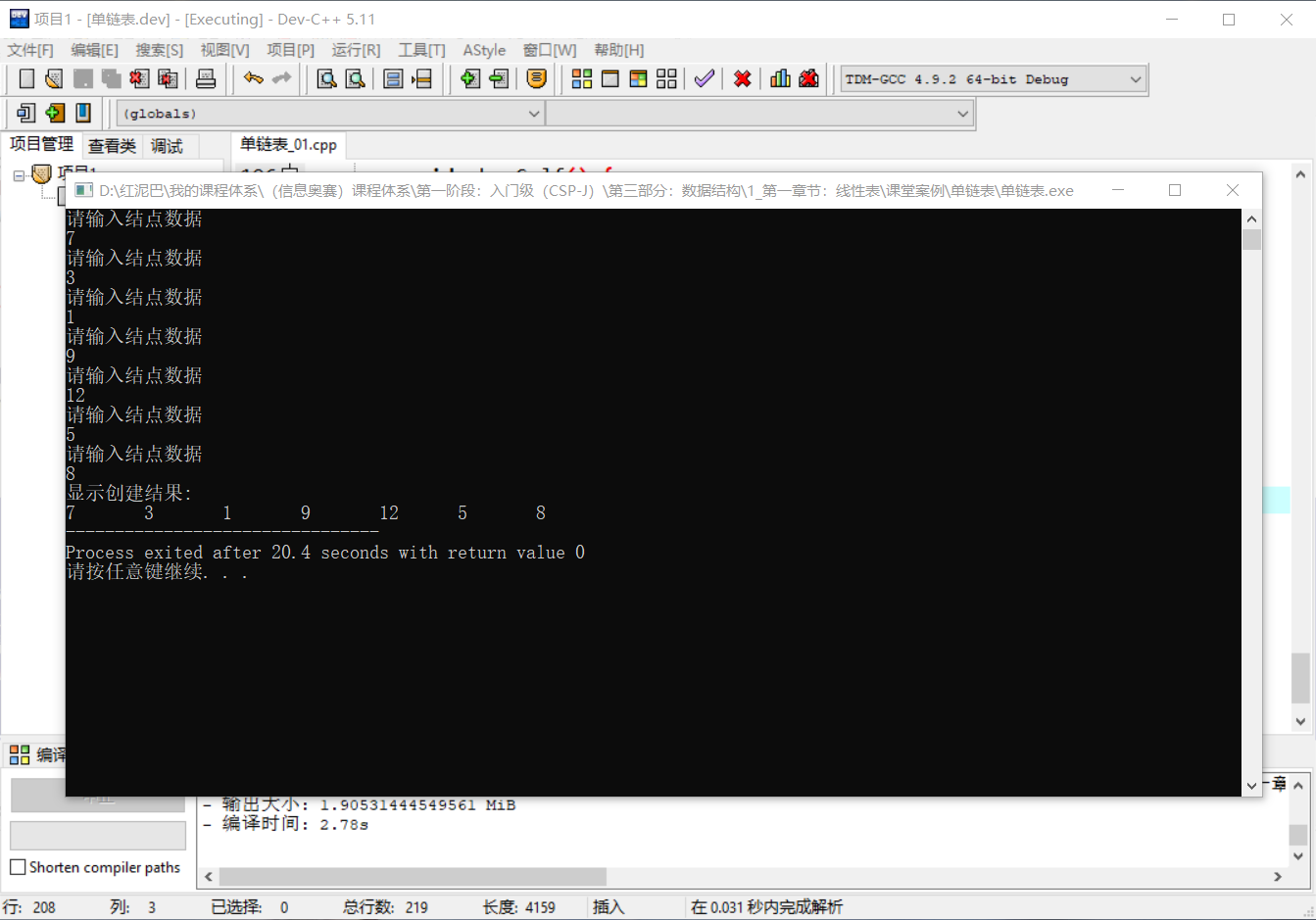• 编写界定算法。
``````void  baseNumBound() {
//个数据结点的数据作为界定数字
//左指针，指向个数据结点
//右指针，指向尾结点

while(left!=right) {
while(left!=right && right->data>tmp) {
//右指针向左移动
right=right->pre;
}
left->data=right->data;
while(left!=right && left->data<tmp) {
//左指针向右移动
left=left->next;
}
right->data=left->data;
}
left->data=tmp;
}
``````

``````int main(int argc, char** argv) {
list.createFromTail(7);
//没删除之前
cout<<"显示链表的创建结果:"<<endl;
list.showSelf();
list.baseNumBound();
cout<<"\n显示界定后的数列："<<endl;
list.showSelf();
return ;
}
``````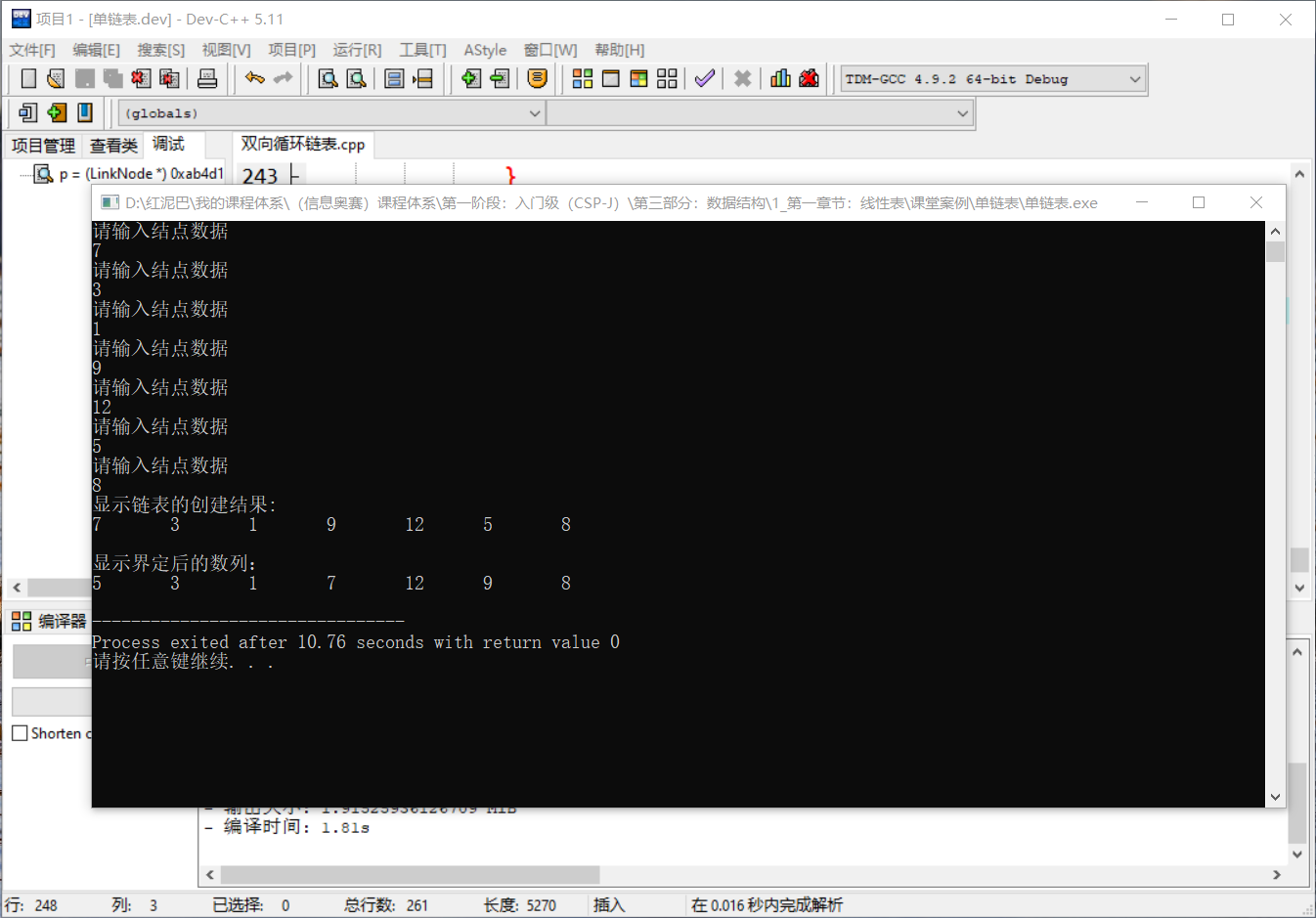## 4. 总结

• 所有用户可根据关注领域订阅专区或所有专区

• 付费订阅：虚拟交易，一经交易不退款；若特殊情况，可3日内客服咨询

• 专区发布评论属默认订阅所评论专区（除付费小栈外）

### 栈主、嘉宾

•jasonhsp
栈主

### 小栈成员

•?
•小尾巴鱼
•karspb
•nt1979